Geosci. Model Dev., 14, 3037–3066, 2021
https://doi.org/10.5194/gmd-14-3037-2021
Geosci. Model Dev., 14, 3037–3066, 2021
https://doi.org/10.5194/gmd-14-3037-2021

Development and technical paper 27 May 2021

Development and technical paper | 27 May 2021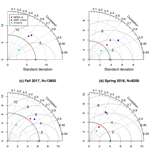# Development and evaluation of CO2 transport in MPAS-A v6.3

Development and evaluation of CO2 transport in MPAS-A v6.3
Tao Zheng1,2, Sha Feng3,a, Kenneth J. Davis3, Sandip Pal4, and Josep-Anton Morguí5,6 Tao Zheng et al.
• 1Department of Geography and Environmental Studies, Central Michigan University, Mount Pleasant, MI, USA
• 2Institute for Great Lakes Research, Central Michigan University, Mount Pleasant, MI, USA
• 3Department of Meteorology and Atmospheric Science, The Pennsylvania State University, University Park, PA, USA
• 4Department of Geosciences, Texas Tech University, Lubbock, TX, USA
• 5Environmental Science and Technology Institute, Universitat Autònoma de Barcelona, ICTA-UAB, Bellaterra, Spain
• 6Department of Evolutionary Biology, Ecology and Environmental Sciences, Universitat de Barcelona, BEECA-UB, Barcelona, Spain
• anow at: Atmospheric Sciences and Global Change Division, Pacific Northwest National Laboratory, Richland, WA, USA

Correspondence: Tao Zheng (zheng1t@cmich.edu)

Abstract

Chemistry transport models (CTMs) play an important role in understanding fluxes and atmospheric distribution of carbon dioxide (CO2). They have been widely used for modeling CO2 transport through forward simulations and inferring fluxes through inversion systems. With the increasing availability of high-resolution observations, it has been become possible to estimate CO2 fluxes at higher spatial resolution. In this work, we implemented CO2 transport in the Model for Prediction Across Scales – Atmosphere (MPAS-A). The objective is to use the variable-resolution capability of MPAS-A to enable a high-resolution CO2 simulation in a limited region with a global model. Treating CO2 as an inert tracer, we implemented in MPAS-A (v6.3) the CO2 transport processes, including advection, vertical mixing by boundary layer scheme, and convective transport. We first evaluated the newly implemented model's tracer mass conservation and then its CO2 simulation accuracy. A 1-year (2014) MPAS-A simulation is evaluated at the global scale using CO2 measurements from 50 near-surface stations and 18 Total Carbon Column Observing Network (TCCON) stations. The simulation is also compared with two global models: National Oceanic and Atmospheric Administration (NOAA) CarbonTracker v2019 (CT2019) and European Centre for Medium-Range Weather Forecasts (ECMWF) Integrated Forecasting System (IFS). A second set of simulation (2016–2018) is used to evaluate MPAS-A at regional scale using Atmospheric Carbon and Transport – America (ACT-America) aircraft CO2 measurements over the eastern United States. This simulation is also compared with CT2019 and a 27 km WRF-Chem simulation. The global-scale evaluations show that MPAS-A is capable of representing the spatial and temporal CO2 variation with a comparable level of accuracy as IFS of similar horizontal resolution. The regional-scale evaluations show that MPAS-A is capable of representing the observed atmospheric CO2 spatial structures related to the midlatitude synoptic weather system, including the warm versus cold sector distinction, boundary layer to free troposphere difference, and frontal boundary CO2 enhancement. MPAS-A's performance in representing these CO2 spatial structures is comparable to the global model CT2019 and regional model WRF-Chem.

Share
1 Introduction

Carbon dioxide (CO2) is the most important greenhouse gas, and our knowledge about its sources and sinks still has large gaps. Inversion systems are tools for inferring surface CO2 fluxes based on observations and chemistry transport models (CTMs). Two types of CTMs are commonly used: global models and regional models. Global models are commonly used for inferring CO2 fluxes at coarse spatial scales . With the fast increasing number of atmospheric CO2 observations, including those acquired by ground-based, airborne, and satellite instruments, regional inversion systems have been developed and applied to estimate carbon fluxes at higher resolution .

A major challenge of atmospheric CO2 inversion modeling is how to partition the model–data mismatch (MDM) among the transport model error, observation error, and prior flux error . In the Bayesian inversion framework, the error covariance matrix R is commonly used to represent the combined error of transport model and observations. While it is important to correctly represent the transport model error in an inversion system, it is also important to reduce the error in order to estimate the fluxes with less uncertainty. One approach to reduce the transport model error is to increase the horizontal resolution of a simulation. For instance, found high-resolution WRF-Chem simulation improved CO2 model–data comparison because of better resolved planetary boundary layer (PBL) and better representation of spatial variability of CO2 fluxes. In a recent study, investigated the impacts of transport model's horizontal resolutions on simulated CO2 accuracy, and they found that CO2 variability is generally better represented by higher-resolution simulations.

Global high-resolution CO2 simulations require large computational resources. Regional (limited-area) models, which have lower computational cost than their global model counterpart at the same horizontal resolution, are often used for high-resolution CO2 transport and inverse modeling . However, a regional model requires CO2 transported from outside its model domain to be prescribed. For a CO2 inversion system, having lateral boundaries increases the size of the control vector to be optimized . A number of approaches have been applied to the CO2 lateral boundary problem, such as assuming the boundary inflow is perfectly known , correcting the lateral boundary condition using observation prior to inversion , or jointly optimizing flux and lateral boundary condition . When the CO2 lateral boundary is optimized, an inversion system adjusts its CO2 fields at the boundary prescribed by a parent global model in addition to adjusting surface fluxes. This could be problematic for inversion systems that use satellite-derived column-averaged CO2 measurements (XCO2) because model–data mismatches in the free troposphere (FT) are often originated from outside a regional model's limited-area domain .

The objective of the present paper is to provide an alternative high-resolution CO2 transport modeling approach to regional transport models. This approach is to use a global variable-resolution model which allows for local grid refinement that enables high-resolution simulation over an interested region without incurring the prohibitively high computational cost or the lateral boundary condition. Variable resolution through local grid refinement has been widely used in numerical weather prediction (NWP) models, such as the Model for Prediction Across Scales – Atmosphere (MPAS-A) , Ocean–Land–Atmosphere Model (OLAM) , Energy Exascale Earth System Model (E3SM) , and Finite-Volume Cubed-Sphere model (FV3) . One benefit of local mesh refinement is enabling regional high-resolution modeling without incurring the lateral boundary condition and its associated problems, such as solution mismatches between the driving global model and the evolving regional model (Davies2014). MPAS-A is a fully compressible non-hydrostatic global atmospheric model which uses finite-volume numeric solver discretized on a centroidal Voronoi mesh with C-grid staggering of its prognostic variables . The centroidal Voronoi mesh allows for local refinement and a variable-resolution horizontal mesh which can be gradually changed from coarse to fine resolutions .

To enable CO2 transport modeling, we implemented atmospheric CO2 transport processes, including advection, vertical mixing by PBL scheme, and convective transport in MPAS-A v6.3. Because the CO2 transport processes are fully integrated into the model's meteorological time steps, the resulting MPAS-A CO2 is an online CTM. We used the newly developed model to conduct two sets of simulations over a 60–15 km variable-resolution global domain. Then the simulation results are evaluated using an extensive set of airborne observations over the eastern United States and near-surface observations from surface and tower stations across the globe. The simulation accuracy of MPAS-A is compared with three established CO2 modeling systems based on the same observational data: WRF-Chem , CarbonTracker (v2019, CT2019 hereafter) , and the European Centre for Medium-Range Weather Forecasts (ECMWF) Integrated Forecasting System (IFS) .

2 Implementation of CO2 transport in MPAS-A

This section describes the major modifications to MPAS-A that we made to implement CO2 tracer transport. We represent CO2 by its dry-air mixing ratio (${q}_{{\mathrm{co}}_{\mathrm{2}}}$) and model its atmospheric transport by adding its continuity equation in MPAS-A following Eq. (7) of :

$\begin{array}{}\text{(1)}& \frac{\partial \left(\stackrel{\mathrm{̃}}{\mathit{\rho }}\phantom{\rule{0.125em}{0ex}}{q}_{{\mathrm{co}}_{\mathrm{2}}}\right)}{\partial t}=-\left(\mathrm{\nabla }\cdot \stackrel{\mathrm{̃}}{\mathit{\rho }}\phantom{\rule{0.125em}{0ex}}{q}_{{\mathrm{co}}_{\mathrm{2}}}\phantom{\rule{0.125em}{0ex}}\mathbit{V}{\right)}_{\mathit{\zeta }}+{F}_{\mathrm{bl}}+{F}_{\mathrm{cu}},\end{array}$

where $\stackrel{\mathrm{̃}}{\mathit{\rho }}={\mathit{\rho }}_{\mathrm{d}}/\left(\partial \mathit{\zeta }/\partial z\right)$, ρd is dry-air density, ζ is the vertical coordinate, z is geometric height, t is time, and $\mathbit{V}=\left(u,v,w\right)$ is the velocity vector (u, v, and w are the zonal, meridional, and vertical wind, respectively). The left-hand side of the equation is the total CO2 time tendency ($\partial \left(\stackrel{\mathrm{̃}}{\mathit{\rho }}{q}_{{\mathrm{co}}_{\mathrm{2}}}\right)/\partial t$), and the first, second, and third terms on the right-hand side represent the contributions from advection, vertical mixing, and convective transport, respectively. CO2 tendency from advection is modeled in flux form (Sect. 2.1), while tendency from vertical mixing (Fbl) and convective transport (Fcu) are modeled in an uncoupled form ($\partial {q}_{{\mathrm{co}}_{\mathrm{2}}}/\partial t\right)$, which are coupled to $\stackrel{\mathrm{̃}}{\mathit{\rho }}$ before being added to the total tendency. We choose to implement CO2 vertical mixing in the Yonsei University (YSU) PBL scheme , and CO2 convective transport in Kain–Fritsch (KF) scheme (Kain2004) because they are widely used in CTMs and have been validated using observations . Details of the three terms on the right-hand side of Eq. (1) are described in the following sections. We note that because the monotonicity constraint in the third-order scalar horizontal advection scheme introduces dissipation, MPAS-A does not use any explicit horizontal diffusion for scalar. Accordingly, we did not include horizontal diffusion for CO2.

## 2.1 CO2 advection

Advection is the most significant component of CO2 atmospheric transport. Following the example of other scalars in MPAS-A , we model CO2 advection as

$\begin{array}{}\text{(2)}& \begin{array}{rl}\left(\mathrm{\nabla }\cdot \stackrel{\mathrm{̃}}{\mathit{\rho }}\phantom{\rule{0.125em}{0ex}}{q}_{{\mathrm{co}}_{\mathrm{2}}}\phantom{\rule{0.125em}{0ex}}\mathbit{V}{\right)}_{\mathit{\zeta }}& ={\left[\frac{\partial \left(\stackrel{\mathrm{̃}}{\mathit{\rho }}u{q}_{{\mathrm{co}}_{\mathrm{2}}}\right)}{\partial x}+\frac{\partial \left(\stackrel{\mathrm{̃}}{\mathit{\rho }}v{q}_{{\mathrm{co}}_{\mathrm{2}}}\right)}{\partial y}\right]}_{\mathit{\zeta }}\\ & +\frac{\partial \left(\stackrel{\mathrm{̃}}{\mathit{\rho }}w{q}_{{\mathrm{co}}_{\mathrm{2}}}\right)}{\partial \mathit{\zeta }}.\end{array}\end{array}$

The first item on the right-hand side enclosed in the square bracket is the CO2 horizontal flux divergence, and second item is the vertical flux divergence. The horizontal flux divergence is transformed via the divergence theorem into an integral of flux over each control volume, which is modeled as

$\begin{array}{}\text{(3)}& {\left[\frac{\partial \left(\stackrel{\mathrm{̃}}{\mathit{\rho }}u{q}_{{\mathrm{co}}_{\mathrm{2}}}\right)}{\partial x}+\frac{\partial \left(\stackrel{\mathrm{̃}}{\mathit{\rho }}v{q}_{{\mathrm{co}}_{\mathrm{2}}}\right)}{\partial y}\right]}_{\mathit{\zeta }}=\frac{\mathrm{1}}{{A}_{i}}\sum _{e}^{{n}_{\mathrm{e}}}\phantom{\rule{0.125em}{0ex}}{l}_{\mathrm{e}}\phantom{\rule{0.125em}{0ex}}{F}_{\mathrm{e}}\left({\mathbit{V}}_{\mathrm{H}},\stackrel{\mathrm{̃}}{\mathit{\rho }}\phantom{\rule{0.125em}{0ex}}{q}_{{\mathrm{co}}_{\mathrm{2}}}\right),\end{array}$

where e indexes the edges of a cell and ne represents the number of edges the cell has, le is the length of an edge, Ai is the cell's areal size, ${F}_{\mathrm{e}}\left({\mathbf{v}}_{\mathrm{H}},\stackrel{\mathrm{̃}}{\mathit{\rho }}\phantom{\rule{0.125em}{0ex}}{q}_{{\mathrm{co}}_{\mathrm{2}}}\right)$ is the instantaneous horizontal CO2 flux that crosses the cell edge e, and ${\mathbit{V}}_{\mathrm{H}}=\left(u,v\right)$ is the horizontal wind vector. The details of MPAS-A instantaneous horizontal flux calculation can found in . The vertical CO2 flux divergence in Eq. (2) is calculated using the finite difference:

$\begin{array}{}\text{(4)}& \frac{\partial \left(\stackrel{\mathrm{̃}}{\mathit{\rho }}w{q}_{{\mathrm{co}}_{\mathrm{2}}}\right)}{\partial \mathit{\zeta }}=\frac{\mathrm{1}}{\mathrm{\Delta }\mathit{\zeta }}\left[F\left(w,\stackrel{\mathrm{̃}}{\mathit{\rho }}\phantom{\rule{0.125em}{0ex}}{q}_{{\mathrm{co}}_{\mathrm{2}}}{\right)}_{k+\frac{\mathrm{1}}{\mathrm{2}}}-F\left(w,\stackrel{\mathrm{̃}}{\mathit{\rho }}\phantom{\rule{0.125em}{0ex}}{q}_{{\mathrm{co}}_{\mathrm{2}}}{\right)}_{k-\frac{\mathrm{1}}{\mathrm{2}}}\right],\end{array}$

where $F\left(w,\stackrel{\mathrm{̃}}{\mathit{\rho }}\phantom{\rule{0.125em}{0ex}}{q}_{{\mathrm{co}}_{\mathrm{2}}}\right)$ is the vertical CO2 flux that crosses a cell's vertical face, and k indexes the vertical coordinate.

## 2.2 CO2 vertical mixing

Like in WRF , a PBL parameterization in MPAS-A treats the vertical mixing of momentum and scalars not only in the boundary layer (BL) but in the entire atmospheric column. The YSU scheme is one of the PBL schemes available in MPAS-A v6.3. The present YSU scheme treats vertical mixing of momentum, potential temperature, and water species but not atmospheric tracers. We modified the scheme to treat CO2 vertical mixing.

In the YSU scheme, after the top of BL is determined, the vertical mixing processes of momentum, potential temperature, and water vapor are treated separately: above the BL, local K-profile approach (Louis1979) is used for vertical diffusion of momentum and scalars . Within the BL, an entrainment flux at the inversion layer is included for momentum and scalar diffusion. In addition, a countergradient mixing term is included for the diffusion of momentum and potential temperature to account for the convective-driven mixing (γc of Eq. 4 in ), but this term is not used for water vapor.

Following the treatment of water vapor, we parameterize CO2 vertical mixing in BL as

$\begin{array}{}\text{(5)}& \frac{\partial {q}_{{\mathrm{co}}_{\mathrm{2}}}}{\partial t}=\frac{\partial }{\partial z}\left[{K}_{\mathrm{h}}\left(\frac{\partial {q}_{{\mathrm{co}}_{\mathrm{2}}}}{\partial z}\right)-\stackrel{\mathrm{‾}}{\left({w}^{\prime }{q}_{{\mathrm{co}}_{\mathrm{2}}}^{\prime }{\right)}_{\mathrm{h}}}\phantom{\rule{0.125em}{0ex}}{\left(\frac{z}{h}\right)}^{\mathrm{3}}\right],\end{array}$

where z is the vertical distance to surface, h is BL top height, Kh is vertical eddy diffusivity. Note that this formulation does not include a countergradient mixing term following the treatment of water vapor in the original YSU scheme . The second term in the square brackets of Eq. (5) represents the contribution from CO2 entrainment flux at the inversion layer, which is parameterized as

$\begin{array}{}\text{(6)}& \stackrel{\mathrm{‾}}{\left({w}^{\prime }{q}_{{\mathrm{co}}_{\mathrm{2}}}^{\prime }{\right)}_{\mathrm{h}}}={w}_{\mathrm{e}}\mathrm{\Delta }{q}_{{\mathrm{co}}_{\mathrm{2}}}{|}_{\mathrm{h}},\end{array}$

where $\mathrm{\Delta }{q}_{{\mathrm{co}}_{\mathrm{2}}}{|}_{\mathrm{h}}$ is the CO2 mixing ratio difference across the inversion layer, and we is the entrainment rate at the inversion layer calculated by Eq. (A11) of . Above BL top, vertical mixing of CO2 is parameterized as

$\begin{array}{}\text{(7)}& \frac{\partial {q}_{{\mathrm{co}}_{\mathrm{2}}}}{\partial t}=\frac{\partial }{\partial z}\left[{K}_{\mathrm{h}}\left(\frac{\partial {q}_{{\mathrm{co}}_{\mathrm{2}}}}{\partial z}\right)\right].\end{array}$

We use the same value for CO2 vertical diffusivity as water vapor. The details of Kh calculation can be found in the Appendix of , and its value is limited between 0.01 and 1000 m2 s−1 to prevent too-weak or too-strong vertical mixing. The term $\partial {q}_{{\mathrm{co}}_{\mathrm{2}}}/\partial t$ from Eq. (5) is coupled with dry-air density before being applied to the continuity equation (Eq. 1).

## 2.3 CO2 convective transport

For convective transport, we modified the KF scheme (Kain2004) to include the CO2 treatment. KF is a mass-flux convection scheme which rearranges mass in an air column using convective updrafts, downdrafts, and environmental mass fluxes. Both the updraft and downdraft entrain from and detrain to the environment, thus altering the vertical profile of an air column's thermodynamic properties. We added the CO2 convective transport as

$\begin{array}{}\text{(8)}& \begin{array}{rl}\frac{\partial {q}_{{\mathrm{co}}_{\mathrm{2}}}}{\partial t}& =\frac{\left({M}_{\mathrm{u}}+{M}_{\mathrm{d}}\right)}{\mathit{\rho }A}\frac{\partial {q}_{{\mathrm{co}}_{\mathrm{2}}}}{\partial z}+\frac{{M}_{\mathrm{ud}}}{M}\left({q}_{{\mathrm{co}}_{\mathrm{2}}}^{\mathrm{u}}-{q}_{{\mathrm{co}}_{\mathrm{2}}}\right)\\ & +\frac{{M}_{\mathrm{dd}}}{M}\left({q}_{{\mathrm{co}}_{\mathrm{2}}}^{\mathrm{d}}-{q}_{{\mathrm{co}}_{\mathrm{2}}}\right),\end{array}\end{array}$

where ${q}_{{\mathrm{co}}_{\mathrm{2}}}$, ${q}_{{\mathrm{co}}_{\mathrm{2}}}^{\mathrm{u}}$, and ${q}_{{\mathrm{co}}_{\mathrm{2}}}^{\mathrm{d}}$ are the CO2 mixing ratio in the environment, updraft, and downdraft, respectively, Mu and Md are the updraft and downdraft mass, respectively, ρ is the environment air density, A is the horizontal area of a cell, M=ρAδz is the mass of environmental air in a grid box, and Mud and Mdd are the detrainment from the updraft and downdraft, respectively.

In the KF scheme, the updraft and downdraft mass and the rates for the entrainment and detrainment are determined by a steady-state plume model and a convective available potential energy (CAPE) closure assumption: 90 % of the existing CAPE should be removed by the convection parameterization . Because the calculation of the updraft and downdraft mass fluxes is related to a cell's horizontal area, the KF scheme may behave differently in different areas of MPAS-A's variable-resolution grid. The updraft source layers are determined by a search from the model's lowest vertical level for a group of consecutive layers that is buoyant and at least 50 hPa deep (Kain2004). The initial value of CO2 mixing ratio in the updraft is modeled as a pressure-weighted average of the source layers:

$\begin{array}{}\text{(9)}& {q}_{{\mathrm{co}}_{\mathrm{2}}}^{\mathrm{u}}=\sum _{k}\frac{\mathit{\delta }{q}_{{\mathrm{co}}_{\mathrm{2}},k}\phantom{\rule{0.125em}{0ex}}\mathit{\delta }{p}_{k}}{\mathit{\delta }{p}_{k}},\end{array}$

where δpk is layer's pressure depth, and ${q}_{{\mathrm{co}}_{\mathrm{2}},k}$ is the layer's CO2 mixing ratio. The CO2 mixing ratio of the updraft is modified by the entrainment of the environmental air through its ascent from its starting level to the cloud top.

$\begin{array}{}\text{(10)}& {q}_{{\mathrm{co}}_{\mathrm{2}}}^{\mathrm{u}}=\frac{{q}_{{\mathrm{co}}_{\mathrm{2}}}^{\mathrm{u}}{M}_{\mathrm{u}}+{q}_{{\mathrm{co}}_{\mathrm{2}}}{M}_{\mathrm{ue}}}{{M}_{\mathrm{u}}+{M}_{\mathrm{ue}}},\end{array}$

where Mue is the updraft entrainment. The initial CO2 mixing ratio of a downdraft (${q}_{\mathrm{co}\mathrm{2}}^{\mathrm{d}}$) is the same as that of the environment (qco2) at the downdraft starting level and it is modified by entrainment through the downdraft descent:

$\begin{array}{}\text{(11)}& {q}_{{\mathrm{co}}_{\mathrm{2}}}^{\mathrm{d}}=\frac{{q}_{{\mathrm{co}}_{\mathrm{2}}}^{\mathrm{d}}{M}_{\mathrm{d}}+{q}_{{\mathrm{co}}_{\mathrm{2}}}{M}_{\mathrm{de}}}{{M}_{\mathrm{d}}+{M}_{\mathrm{de}}},\end{array}$

where Mde is the downdraft entrainment.

3 Model evaluation

In this section, we evaluate the newly developed MPAS-A CO2 transport model by comparing its simulation results with observations and other models. After describing the simulation configuration (Sect. 3.1), we assess the model's global mass conservation property (Sect. 3.2). Then we evaluate the model's CO2 transport accuracy at the global scale using hourly near-surface CO2 observations from 50 in situ stations and column-averaged CO2 dry-air mole fraction (XCO2) measurements from 18 Total Carbon Column Observing Network (TCCON) stations (Sect. 3.3). Finally, we evaluate MPAS-A at the regional scale using high-resolution airborne measurements from the Atmospheric Carbon and Transport (ACT) campaign over the eastern United States (Sect. 3.4). MPAS-A CO2 transport is also compared with three established CTMs: NOAA CT2019 , ECMWF IFS , and WRF-Chem . In the following model evaluation, we use root mean square error (RMSE), bias (μ), and random error (SE) as the model accuracy metrics:

$\begin{array}{}\text{(12)}& \text{RMSE}=\sqrt{\frac{\mathrm{1}}{N}\sum _{i=\mathrm{1}}^{N}\left({m}_{i}-{o}_{i}{\right)}^{\mathrm{2}}}\text{(13)}& \mathit{\mu }=\frac{\mathrm{1}}{N}\sum _{i=\mathrm{1}}^{N}\left({m}_{i}-{o}_{i}\right)\text{(14)}& \text{SE}=\sqrt{\frac{\mathrm{1}}{N}\sum _{i=\mathrm{1}}^{N}\left({m}_{i}-{o}_{i}-\mathit{\mu }{\right)}^{\mathrm{2}}},\end{array}$

where oi and mi represent the observed and modeled values, respectively.

For model–data intercomparison, MPAS-A model data need to be interpolated to the observation space. Following , the model is sampled in the horizontal by taking the nearest cell over land. MPAS-A uses a height-based terrain-following vertical coordinate . At a given cell, the height of the kth vertical layer boundary is denoted as ${z}_{k}^{\mathrm{h}}$. The height of the layer center is ${z}_{k}=\mathrm{0.5}×\left({z}_{k}^{\mathrm{h}}+{z}_{k+\mathrm{1}}^{\mathrm{h}}\right)$. In MPAS-A, horizontal wind fields are defined at the vertical layer boundaries and CO2 fields are defined at layer centers. For horizontal wind field validations using radiosonde data (Sect. 3.3.1), the column profiles of air pressure and horizontal wind fields defined at layer boundaries are used to interpolate to the measurements' pressure levels. To compare with near-surface CO2 observations from in situ stations (Sect. 3.3.3) and aircraft observations (Sect. 3.4), model CO2 defined at layer centers is interpolated to the measurement heights. Vertical interpolation and integration for the comparison with TCCON XCO2 are described in Sect. 3.3.4. MPAS-A simulation outputs are saved at 1 h intervals. For comparison with radiosonde observations and near-surface CO2 observations, no temporal interpolations are applied: observations are paired with the closest hourly MPAS-A output. For comparison with aircraft observations, the hourly model outputs that bracket an observation's time stamp are used for the temporal interpolation.Figure 1Variable-resolution 60–15 km global domain for MPAS-A CO2 simulations conducted for model evaluation using aircraft and near-surface CO2 observations. The highest-resolution (15 km) grid covering the most of North America has a cell size of less than 250 km2. The cell sizes (represented by color) gradually increase to about 3600 km2 for the rest of the global domain.

## 3.1 Simulation experiment configuration

For all subsequent simulations, MPAS-A uses a 60–15 km variable-resolution global mesh. Figure 1 shows the cell size (in km2) of the simulation domain, where the highest resolution (15 km) over North America has a cell size smaller than 250 km2, which gradually increases to about 3600 km2 for the rest of the global domain. In the vertical direction, there are 55 levels spanning from the surface to 30 km above the mean sea level. The model time step is 90 s, which is in accordance with the highest (15 km) horizontal resolution. For physical parameterizations, in addition to the modified YSU PBL and Kain–Fritsch cumulus schemes (Kain2004) described in Sect. 2, we use RRTMG for longwave and shortwave radiation , the Noah land scheme , the Monin–Obukhov surface layer scheme, and the WRF single-moment six-class microphysics scheme . Third-order accuracy advection is used for all scalars and the CO2 tracer. A summary of the physics parameterizations used in the simulations is given in Table 1.

Initial meteorological fields are generated from the ERA-Interim reanalysis . To keep model meteorological fields close to the reanalysis, MPAS-A meteorological fields are re-initialized using the analysis at 00:00 UTC each day throughout a simulation period. The CO2 mixing ratio is kept unchanged during the meteorology re-initializations; thus, it is a free-running simulation. This configuration is the same as that used by in their IFS global CO2 simulations. The first CO2 initial condition for a simulation is from the CT2019 3× 2 posterior dry-air mole fraction product and surface CO2 fluxes are prepared by interpolating the CT2019 3-hourly 1× 1 posterior flux product . The four components of CT2019 fluxes (biosphere, ocean, fossil fuel, and fire) are interpolated to the MPAS-A model grid and ingested at 3 h intervals throughout a simulation.

## 3.2 CO2 mass conservation

For CTMs, it is very important to maintain the global CO2 mass conservation . Because meteorological re-initializations introduce changes in dry-air mass, they impact MPAS-A's global CO2 mass conservation. We first examine MPAS-A's inherent mass conservation property through a simulation without the meteorological re-initializations in Sect. 3.2.1. Then we examine and treat the impacts of the meteorological re-initializations in Sect. 3.2.2.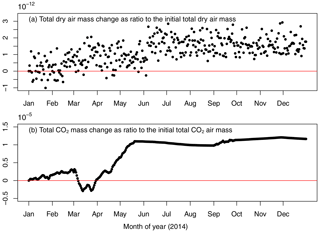Figure 2Variation of total dry-air mass (a) and total CO2 mass (b) as the ratio to their respective starting values during a 1-year continuous MPAS-A simulation without meteorological re-initialization. The x axis represents the days of 2014 and the y axis the ratio of the total mass change to the starting values.

### 3.2.1 Mass conservation without meteorology re-initialization

To examine MPAS-A's mass conservation property, we conducted a MPAS-A simulation that lasts from 1 January to 31 December 2014. The simulation is initialized with the CT2019 CO2 mole fraction and is driven with 3-hourly CT2019 surface CO2 fluxes. Meteorological re-initializations are not applied during the simulation and the model outputs are saved using double precision. MPAS-A's global dry-air mass (Mair) is then calculated at 00:00 UTC each day through the 1-year simulation using Eq. (15):

$\begin{array}{}\text{(15)}& {\mathrm{M}}_{\mathrm{air}}=\sum _{k}^{L}\left(\sum _{i}^{N}{A}_{i}\phantom{\rule{0.125em}{0ex}}{h}_{i,k}\phantom{\rule{0.125em}{0ex}}{\mathit{\rho }}_{i,k}\right),\end{array}$

where subscript i indexes the horizontal cell, subscript k indexes the vertical level, Ai is cell size, hi,k is cell height, and ρi,k is dry-air density (kg m−3). After the model's global dry-air mass is calculated at 00:00 UTC each day of the simulation period, its variation is quantified as a ratio ${E}_{\mathrm{air}}^{t}=\left({M}_{\mathrm{air}}^{t}-{M}_{\mathrm{air}}^{\mathrm{0}}\right)/{M}_{\mathrm{air}}^{\mathrm{0}}$, where ${M}_{\mathrm{air}}^{\mathrm{0}}$ and ${M}_{\mathrm{air}}^{t}$ are the model's global dry-air mass at the simulation start (00:00 UTC, 1 January 2014) and the current time step, respectively. The top panel of Fig. 2 shows ${E}_{\mathrm{air}}^{t}$ at 00:00 UTC of each day through the 1-year simulation period. The figure shows that the maximal magnitude of ${E}_{\mathrm{air}}^{t}$ is less than $\mathrm{4}×{\mathrm{10}}^{-\mathrm{12}}$ during the 1-year simulation. In comparison, the total dry-air mass of ECMWF IFS increases by about 0.01 % of its initial value in a 10 d forecast . Similarly, the Environment and Climate Change Canada (ECCC) Global Environmental Multiscale (GEM-MACH-GHG) model loses about 0.01 % of its initial total dry-air mass in a 10 d forecast . MPAS-A has a significantly lower global dry-air mass variation than the two global models because its explicit grid point advection scheme conserves mass , while the semi-Lagrangian advection scheme used by IFS and GEM-MACH-GHG does not conserves mass . Thus, no mass fixer is used in MPAS-A.

MPAS-A's global CO2 mass (${M}_{{\mathrm{co}}_{\mathrm{2}}}$) is calculated using Eq. (16):

$\begin{array}{}\text{(16)}& {M}_{{\mathrm{co}}_{\mathrm{2}}}=\sum _{k}^{L}\left(\sum _{i}^{N}{A}_{i}\phantom{\rule{0.125em}{0ex}}{h}_{i,k}\phantom{\rule{0.125em}{0ex}}{\mathit{\rho }}_{i,k}\phantom{\rule{0.125em}{0ex}}{q}_{i,k}\right),\end{array}$

where qi,k is the CO2 dry-air mixing ratio ($\mathrm{kg}/\mathrm{kg}$) and the rest of the terms are the same as in Eq. (15). To assess the global CO2 mass conservation, ${M}_{{\mathrm{co}}_{\mathrm{2}}}$ calculated using Eq. (16) is adjusted for the CO2 mass introduced through the ingestion of the 3-hourly surface CO2 fluxes. For a 3 h period, total CO2 mass introduced through the surface CO2 fluxes is ${\sum }_{i}^{N}{A}_{i}\phantom{\rule{0.125em}{0ex}}{F}_{i}\phantom{\rule{0.125em}{0ex}}\mathrm{\Delta }t$, where Fi is the combined biosphere, ocean, fossil fuel, and fire CO2 fluxes ($\mathrm{kg}\phantom{\rule{0.125em}{0ex}}{\mathrm{m}}^{-\mathrm{2}}\phantom{\rule{0.125em}{0ex}}{\mathrm{s}}^{-\mathrm{1}}$) at a surface cell, Ai is the cell's areal size, N is number of surface cell, and Δt=3 h. After the adjustment, the variation of global mass of CO2 is quantified as a ratio, ${E}_{{\mathrm{co}}_{\mathrm{2}}}^{t}=\left({M}_{{\mathrm{co}}_{\mathrm{2}}}^{t}-{M}_{{\mathrm{co}}_{\mathrm{2}}}^{\mathrm{0}}\right)/{M}_{{\mathrm{co}}_{\mathrm{2}}}^{\mathrm{0}}$, where ${M}_{{\mathrm{co}}_{\mathrm{2}}}^{\mathrm{0}}$ and ${M}_{{\mathrm{co}}_{\mathrm{2}}}^{t}$ are the global CO2 mass at the initial and current time step, respectively. ${E}_{{\mathrm{co}}_{\mathrm{2}}}^{t}$ at 00:00 UTC of each day of the simulation period is shown in the lower panel of Fig. 2. The figure shows that the maximal magnitude of ${E}_{{\mathrm{co}}_{\mathrm{2}}}^{t}$ is about 10−5. This is much higher compared to ${E}_{\mathrm{air}}^{t}$ and it is due to the strong gradients caused by surface CO2 flux which challenge the model's numerical scheme.

### 3.2.2 CO2 mass conservation during meteorology re-initialization

When meteorological re-initialization is applied during a simulation, the values of dry-air density in MPAS-A are replaced by values from the initialization files generated from the ERA-Interim reanalysis. In most cases, this will cause dry-air density change which in turn will introduce CO2 mass change if CO2 dry-air mixing ratios are kept unchanged during the re-initialization. To assess this possible change in global CO2 mass, we conducted another 1-year long MPAS-A simulation identical to that used in Sect. 3.2.1, except that meteorological re-initialization was applied at 24 h intervals during the simulation. The variation of global CO2 mass caused by a meteorological re-initialization is quantified as a ratio $E=\left({M}_{{\mathrm{co}}_{\mathrm{2}}}^{\prime }-{M}_{{\mathrm{co}}_{\mathrm{2}}}\right)/{M}_{{\mathrm{co}}_{\mathrm{2}}}$, where ${M}_{{\mathrm{co}}_{\mathrm{2}}}$ and ${M}_{{\mathrm{co}}_{\mathrm{2}}}^{\prime }$ are the global CO2 mass before and after a meteorological re-initialization. The top panel of Fig. 3 shows the value of E at each meteorological re-initialization. The figure indicates that a meteorological re-initialization could cause a change of more than 0.01 % of the global CO2 mass.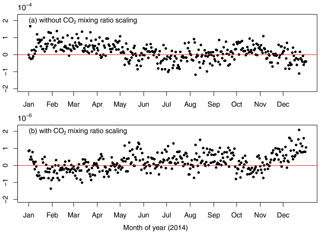Figure 3Variation of global CO2 mass as the ratio to its value prior to a meteorological re-initialization during a 1-year MPAS-A simulation with meteorological re-initializations at 24 h intervals. The top panel is from the simulation without applying CO2 mixing ratio scaling as described in Sect. 3.2.2, and the bottom panel is from the simulation with the scaling. In each figure, the x axis represents the days of 2014 and the y axis the ratio of global CO2 mass variation to its value prior to a meteorological re-initialization.

To keep the CO2 mass conservation after a meteorological re-initialization, we adjust MPAS-A's CO2 fields by a spatially uniform scaling factor: ${q}_{i,k}^{\prime }=r×{q}_{i,k}$, where qi,k and ${q}_{i,k}^{\prime }$ are the CO2 dry-air mixing ratio, before and after the adjustment, respectively. The scaling factor r is calculated as

$\begin{array}{}\text{(17)}& r=\frac{{\sum }_{k}^{L}\left({\sum }_{i}^{N}{A}_{i}\phantom{\rule{0.125em}{0ex}}{h}_{i,k}\phantom{\rule{0.125em}{0ex}}{\mathit{\rho }}_{i,k}\phantom{\rule{0.125em}{0ex}}{q}_{i,k}\right)}{{\sum }_{k}^{L}\left({\sum }_{i}^{N}{A}_{i}\phantom{\rule{0.125em}{0ex}}{h}_{i,k}\phantom{\rule{0.125em}{0ex}}{\mathit{\rho }}_{i,k}^{\prime }\phantom{\rule{0.125em}{0ex}}{q}_{i,k}\right)},\end{array}$

where the notations are the same as in Eq. (16) except that ${\mathit{\rho }}_{i,k}^{\prime }$ is the dry-air density after a meteorology re-initialization and ρi,k is the value before the re-initialization. To test the effectiveness of this scaling method, the 1-year MPAS-A simulation with meteorological re-initialization was conducted again but this time with the CO2 dry-air mixing ratio adjustment applied after each meteorological re-initialization. The resulting variation in total CO2 mass is plotted in the lower panel of Fig. 3. The figure shows the maximal magnitude of the variation caused by a meteorological re-initialization has been reduced from $\sim {\mathrm{10}}^{-\mathrm{4}}$ to $\sim {\mathrm{10}}^{-\mathrm{6}}$ of the global CO2 mass. Note the different scales in the y axis used in the top and bottom panels of Fig. 3.

An alternative approach to restore mass conservation is to scale the CO2 mixing ratio at each grid box individually by

$\begin{array}{}\text{(18)}& {q}_{i,k}^{\prime }=\frac{{\mathit{\rho }}_{i,k}}{{\mathit{\rho }}_{i,k}^{\prime }}×{q}_{i,k},\end{array}$

where the notation is the same as that in Eq. (17). This scaling approach can maintain global CO2 mass conservation as allowed by machine precision but it will introduce artificial spatial variations in CO2 mixing ratio. In the simulations in the following sections, we chose to use the first scaling approach to avoid the artificial CO2 mixing ratio variation by accepting the small changes in global CO2 mass.

## 3.3 Model evaluation at global scale

In this section, we evaluate the MPAS-A CO2 transport at the global scale. For the model evaluation, MPAS-A was initialized at 00:00 UTC on 1 July 2013 and ran until 31 December 2014. The model configuration for this simulation is as described in Sect. 3.1. With the first 6-month as model spin-up, we use the 1-year simulation of 2014 for the model evaluation. First, MPAS-A simulated horizontal wind fields are evaluated using radiosonde measurements from 457 stations. Then, the model's CO2 fields are compared with CT2019, near-surface CO2 measurements from 50 stations, and XCO2 retrievals from 18 TCCON stations.

### 3.3.1 Evaluation of horizontal wind fields

Accurate meteorological fields are critical for an accurate CO2 transport simulation. Before evaluating the simulated CO2, we evaluate the MPAS-A simulated horizontal wind fields considering their importance in CO2 advection. We compare MPAS-A simulated horizontal wind fields at 12:00 and 00:00 UTC each day of the simulation period with radiosonde observations from 457 stations located around the globe at four pressure levels: 1000, 850, 500, 850, and 200 hPa. Note that because of the 24-hourly meteorological re-initialization, the 00:00 and 12:00 UTC simulation results are 12 and 24 h forecasts, respectively. The locations of the 457 radiosonde stations are shown in Fig. S1 of the Supplement.

Table 2Evaluation of MPAS-A simulated horizontal wind using NOAA Integrated Global Radiosonde Archive (IGRA) radiosonde data. Radiosonde data from 457 stations over the globe are used for the evaluation. Wind speed and direction are compared at 00:00 and 12:00 UTC at four pressure levels (1000, 850, 500, and 200 hPa) for each day of the MPAS-A simulation. The number of data samples (N) is smaller at 1000 hPa because some stations are located above that pressure level.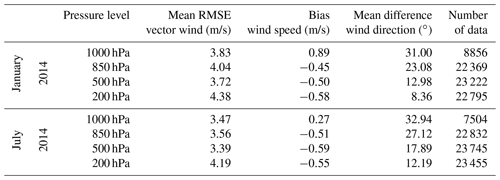To compare with the similar validation results reported in , the horizontal wind field evaluation results for January and July of 2014 are listed in Table 2. The table shows that while the mean difference in wind direction decreases with altitude, the mean RMSE vector wind generally increases with altitude, which agrees with the IFS validation results . At the 1000 hPa level, MPAS-A has a slightly lower accuracy than IFS during the same time period. For instance, MPAS-A's mean RMSE vector wind at 1000 hPa is 3.83 m s−1 for January 2014, and IFS results range from 3.2 to 3.75 m s−1 for its 9 and 80 km horizontal resolution simulations. For July 2014, the mean RMSE vector wind at 1000 hPa is 3.47 m s−1 from MPAS-A and 3.0 to 3.6 m s−1 for the IFS 9 and 80 km simulations. At upper level, MPAS-A has a slightly higher accuracy than IFS: at 500 hPa, MPAS-A mean RMSE vector wind is 3.72 and 3.39 m s−1 for January and July 2014, respectively, while IFS results in 4.0–4.1 and 3.5–3.6 m s−1 for the same time period.

Table 3The statistics of MPAS-A simulated horizontal wind validated at radiosonde stations located in North America. In each cell, the first value is from the 60–15 km variable-resolution grid simulation (labeled as 15 km) and the second is from the 60 km uniform grid simulation (labeled as 60 km). Note that the number of data at 850 and 1000 hPa is different between the two simulations because of the differences in their grids' topography.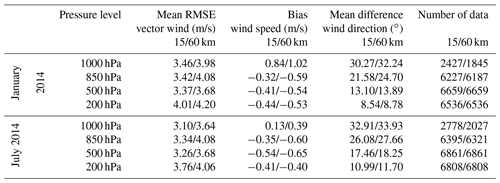An important finding of is that higher horizontal resolution generally leads to higher meteorological and CO2 simulation accuracy. To examine the influence of horizontal resolution on MPAS-A's meteorological simulation accuracy, we conducted an additional set of simulations using the identical configuration except with a global 60 km uniform-resolution grid instead of the 60–15 km variable-resolution grid (Fig. 1). Out of the 475 radiosonde stations, 131 are located in 15 km cells in the 60–15 km variable-resolution simulation. These 131 radiosonde stations are all located at 60 km cells in the 60 km uniform-resolution simulation. In Table 3, we calculated and compared horizontal wind accuracy at these 131 radiosonde stations between the 60 km uniform-resolution simulation (labeled as 60 km) and the 60–15 km variable-resolution simulation (labeled as 15 km). The table shows that the horizontal wind fields at these 131 stations are simulated with considerably higher accuracy on the 15 km grid than its 60 km grid counterpart. For instance, at 1000 hPa, the mean RMSE wind vector for January 2014 is 3.46 and 3.98 m s−1 at the 15 and 60 km grids, respectively. The values are 3.10 and 3.64 m s−1 for July 2014. Table 3 also shows that the difference in the mean RMSE wind vector between the 15 and 60 km grids is larger near the surface at 850 and 1000 hPa than in the middle and upper troposphere (500 and 200 hPa), which is consistent with the findings of . For both January and July at the four pressure levels, the mean RMSE wind vector at the 131 radiosonde stations in MPAS-A's 15 km grid is either similar to or slightly lower than the mean RMSE wind vector of the around 400 stations from the IFS 9 km resolution simulation .

Table 4Continuous in situ stations used for evaluating MPAS-A CO2 simulation accuracy. NA denotes references that are not available.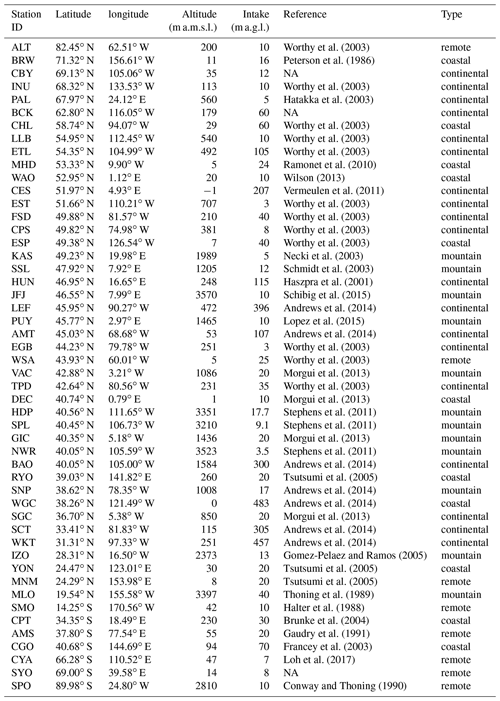### 3.3.2 Comparison of CO2 fields with CarbonTracker

Having established that the horizontal wind fields simulated by MPAS-A are sufficiently accurate, the CO2 fields can be evaluated. Here, we directly compare the simulated XCO2 by MPAS-A and CT2019 at the grid scale. CT2019 is an operational carbon data-assimilation system which uses Transport Model 5 (TM5) for atmospheric transport. TM5 is an offline global CTM which includes CO2 advection, deep and shallow convection, and vertical diffusion in both PBL and FT . In producing the CT2019 CO2 mole fraction , the TM5 simulation ran over a 3× 2 global domain.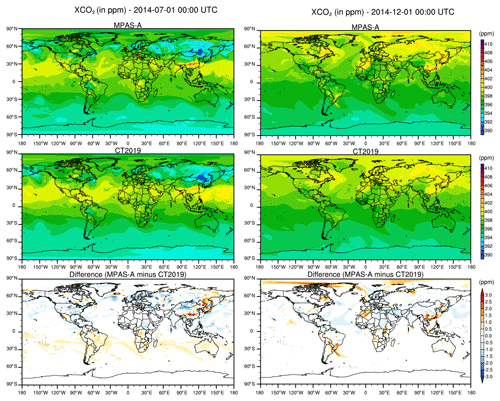Figure 4Simulated XCO2 of MPAS-A, CT2019, and their difference on 1 July 2014 and 1 December 2014, 00:00 UTC.

First, XCO2 values are calculated on the native grid for MPAS-A (60–15 km) and CT2019 (3× 2). XCO2 at a given model cell is calculated as the pressure-weighted CO2 dry-air mixing ratio:

$\begin{array}{}\text{(19)}& {\mathrm{XCO}}_{\mathrm{2}}=\left(\sum _{k=\mathrm{1}}^{N}{p}_{k}{q}_{k}^{{\mathrm{co}}_{\mathrm{2}}}\right)/\left(\sum _{k=\mathrm{1}}^{N}{p}_{k}\right),\end{array}$

where pk is modeled air pressure at layer k corrected for water vapor, ${q}_{k}^{{\mathrm{co}}_{\mathrm{2}}}$ is CO2 dry-air mole fraction at the same level. N is the number of vertical levels in a model. Then, XCO2 values from MPAS-A and CT2019 are regridded from their respective grids on an identical 1×1 grid for a direct comparison. Figure 4 shows the comparison of XCO2 from MPAS-A (top) and CT2019 (middle) and their difference (bottom) for 1 July and 1 December 2014 at 00:00 UTC. The figure shows that XCO2 values from MPAS-A and CT2019 are generally consistent at large scales, but differences exist at small spatial scales. For instance, the difference in horizontal resolution between MPAS-A and CT2019 can be clearly observed in XCO2 in July over both northeastern and southern China. In December, MPAS-A has a higher XCO2 than CT2019 within the Arctic Circle and southern China. Overall, the differences between MPAS-A and CT2019 are evident. The magnitude of differences is mostly within 3 ppm, which is similar to the magnitude reported in for the GEM-MACH-GHG model. Because both models used the same surface CO2 fluxes, the difference in the simulated CO2 fields is only caused by the different model transport: spatial resolution, dynamics, and physical parameterizations. The differences between MPAS-A and CT2019 are expected due to the differences in the two models' horizontal resolution, dynamics, and physical parameterizations. Because no CTM can be expected to have perfect transport, the acceptability of transport is generally judged through comparisons of model simulation with measurements.

### 3.3.3 Comparison with near-surface CO2 measurements

This section compares MPAS-A simulated CO2 with hourly measurements from 50 stations that were used for the IFS model evaluation in . The information of the 50 stations, including location, elevation, intake height, reference, and type, is listed in Table 4. Like in , only the highest intake level is used at towers that have multiple intake heights. When multiple observations within an hour are available (such as those with a 30 min or shorter time interval), they are averaged to yield a single hourly value. For a given station, this results in 744 (24×31) hourly measurements per month at the maximum.

The MPAS-A hourly CO2 statistics, including RMSE, SE, and bias at the 50 stations, are listed in Tables S1 and S2 of the Supplement for January and July 2014, respectively. For comparison, Tables S1 and S2 also include the statistics from the IFS 9 and 80 km resolution simulations at the same sites for the same time periods. Table S1 shows that RMSE of the MPAS-A simulated hourly CO2 ranges from 0.17 ppm at the SPO station to 16.65 ppm at the KAS station. In comparison, the IFS simulations also resulted in a much lower RMSE at the SPO than KAS, the latter of which has a RMSE of 4.44 ppm from the 9 km resolution simulation and 10.71 ppm from the 80 km simulation.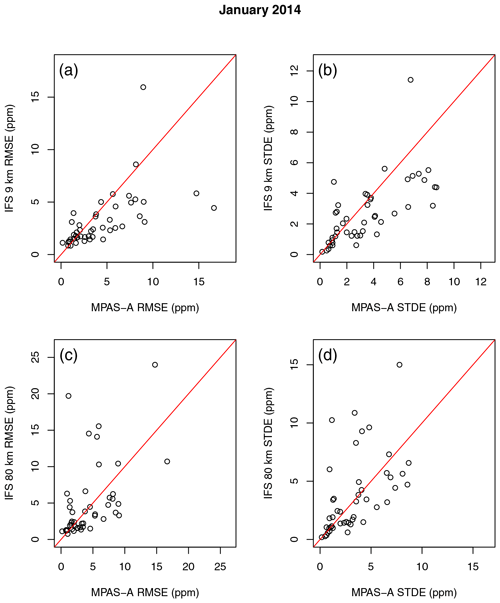Figure 5Comparison of model-simulated hourly CO2 accuracy (RMSE and SE) between MPAS-A and IFS at 50 surface and tower stations. Each open circle in the figures represents a station. Comparisons of MPAS-A with the IFS 9 km resolution simulations are in panels (a) and (b), and comparisons with IFS 80 km resolutions simulations are in panels (c) and (d).

Table 5Comparison of mean RMSE of hourly CO2 from MPAS-A and IFS 9 km and 80 km simulations. p values of the paired t test between MPAS-A and the IFS simulations are also listed.The comparisons of RMSE and SE from MPAS-A and IFS are shown in Figs. 5 and 6 for January and July 2014, respectively. Table 5 uses a paired t test to provide a quantitative summary of the hourly CO2 RMSE between MPAS-A and the IFS 9 and 80 km simulations. The table shows that for January 2014, the mean RMSE at the 50 stations is 4.20 ppm from MPAS-A, which is higher than the IFS 9 km simulation (3.12 ppm, p=0.01) and similar to the IFS 80 km simulation (4.94 ppm, p=0.25). For July 2014, the mean RMSE at the 50 stations is 8.09 ppm from MPAS-A, which is similar to the IFS 9 km simulation (8.04, p=0.95) and lower than the IFS 80 km simulation (11.77 ppm, p=0.04). The above comparisons indicate that the 60–15 km MPAS-A simulation has a level of accuracy between the IFS 9 and 80 km simulations.

Table 6Comparison of RMSE of hourly CO2 between the MPAS-A 60–15 km simulation and the IFS 9 km and 80 km simulations at 12 mountain sites (Table 4). The left half of the table is for six mountain sites located in MPAS-A's 15 km cells and the second half is for six mountain sites located in MPAS-A's 60 km cells. The top half of the table is for January 2014 and the bottom half is for July 2014.found that atmospheric CO2 transport is generally better represented at higher horizontal resolutions, and mountain stations display the largest improvement at higher resolution as they directly benefit from the more realistic orography. There are 12 mountain stations of the 50 stations used for the model validation. Table 6 lists the 12 mountain stations in two groups: the first group includes the six mountain stations located in the 15 km cells of the MPAS-A's 60–15 km variable-resolution grid, and the second group includes the other six stations that are located in the 60 km cells of the grid. The table lists the hourly CO2 RMSE for each of the 12 stations from MPAS-A and IFS 9 and 80 km simulations for January and July 2014. The table shows that at each of the six mountain stations located in 15 km cells, MPAS-A has lower hourly CO2 RMSE than the IFS 9 km simulation for July 2014. For January 2014, MPAS-A has a lower RMSE than the IFS 9 km simulation at five out the six stations (the exception is NWR). In comparison, at the six mountain stations located in its 60 km cells, MPAS-A has higher hourly CO2 RMSE than the IFS 9 km simulation for both January and July 2014 with the exception of JFJ for July 2014.

### 3.3.4 Comparison with TCCON XCO2 measurements

After the comparison with the near-surface CO2 in the last section, we evaluate MPAS-A CO2 fields using XCO2 measurements from 18 TCCON sites listed in Table 7. To compare with TCCON-retrieved XCO2, smoothed MPAS-A XCO2 is calculated following :

$\begin{array}{}\text{(20)}& {X}_{{\mathrm{CO}}_{\mathrm{2}}}^{\mathrm{model}}={c}_{\mathrm{a}}+{\mathbf{h}}^{T}{\mathbf{a}}^{T}\left({\mathbf{x}}_{\mathrm{m}}-{\mathbf{x}}_{\mathrm{a}}\right),\end{array}$

where ${X}_{{\mathrm{CO}}_{\mathrm{2}}}^{\mathrm{model}}$ is the smoothed MPAS-A XCO2, ca is the a priori total column, aT is TCCON column averaging kernel, hT is a dry-pressure weighting function, xm is MPAS-A CO2 dry-air mole fraction profile, xa is the a priori CO2 dry-air mole fraction profile. The column profiles of CO2, air pressure, and water vapor mixing ratio extracted from MPAS-A hourly output are interpolated to the same vertical grid as xa, and the dry-pressure weighting function hT is calculated following and Eq. (A7) of .

At a given TCCON site, averaged hourly XCO2 (denoted as ${X}_{{\mathrm{CO}}_{\mathrm{2}}}^{\mathrm{TCCON}}$) is calculated as the mean value of all valid XCO2 retrievals within the hour. ${X}_{{\mathrm{CO}}_{\mathrm{2}}}^{\mathrm{TCCON}}$ are then matched with the calculated hourly XCO2 from MPAS-A (denoted as ${X}_{{\mathrm{CO}}_{\mathrm{2}}}^{\mathrm{model}}$). The comparisons of ${X}_{{\mathrm{CO}}_{\mathrm{2}}}^{\mathrm{model}}$ and ${X}_{{\mathrm{CO}}_{\mathrm{2}}}^{\mathrm{TCCON}}$ at the 18 TCCON sites for the year 2014 are shown in Fig. 7. The results indicate that the observed seasonal variations in TCCON XCO2 are in general well represented by MPAS-A. The hourly average XCO2 comparisons between MPAS-A and TCCON are summarized in Table 8. In the table, N is the number of data pairs used for calculating the statistics, including RMSE, bias, and correlation coefficient R. The mean RMSE of the 18 sites is 1.35 ppm, which is comparable to the IFS simulations (1.02 to 1.25 ppm) . We then calculated the average daily XCO2 as the mean value of all the hourly XCO2 within a given day. The statistics of the comparison of daily XCO2 between MPAS-A and TCCON are also included in Table 8. In the table, N is the number of average daily XCO2 used for calculating the statistics. Compared to their hourly counterparts, the average daily XCO2 has both lower RMSEs and higher correlation coefficients. The mean value of the average daily XCO2 RMSE of the 18 TCCON sites is 1.23 ppm, which is comparable to IFS simulations (0.97 to 1.25 ppm) reported in .

Table 8Statistics for the average hourly XCO2 and average daily XCO2 comparison between TCCON measurements and MPAS-A simulations: RMSE (ppm), bias (ppm), and correlation coefficient R. N is the number of data pairs used for computing the statistics.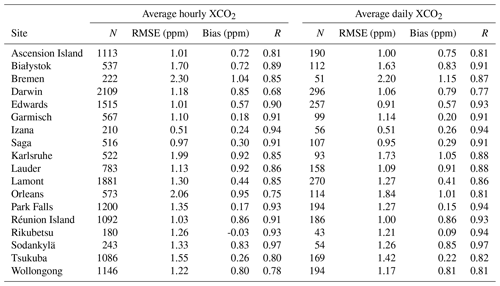## 3.4 Model evaluation at regional scale

In this section, we present an evaluation of the MPAS-A CO2 simulation accuracy using extensive high-resolution CO2 observation data acquired through the ACT aircraft campaigns. ACT is a National Aeronautics and Space Administration (NASA) Earth Venture Suborbital 2 (EVS-2) mission, and its goal is to improve atmospheric inversion estimates of CO2 and CH4 through extensive airborne measurements over the eastern United States during multiple seasons . Through four campaign seasons from summer 2016 to spring 2018 with two research aircraft (C130 and B200), the ACT project collected an extensive dataset of highly resolved CO2 measurements in both the BL and FT. The duration of the ACT campaign seasons is given in Table 9. To use ACT airborne CO2 measurements for model evaluation, we conducted a MPAS-A simulation from 1 January 2016 to 31 May 2018. The first 6 months are for the model spin-up. The simulation uses the domain and configurations as described in Sect. 3.1, and model outputs are saved at 1 h intervals.

First, we compare MPAS-A simulated horizontal wind fields during the ACT campaign seasons using the same procedure described in Sect. 3.3.1. Table 10 lists the statistics of horizontal wind field evaluations during the four ACT campaign seasons. The table indicates the same pattern as in 2014 (Table 2): the mean RMSE vector wind increases with altitude and the mean difference of wind direction decreases with altitude. The magnitude of the statistics of the four ACT campaign seasons is comparable to that of 2014 (Table 2).

Table 10Evaluation of the simulated horizontal wind of MPAS-A using radiosonde observations at 457 stations located across the globe. Wind speed and direction are compared at 00:00 and 12:00 UTC at four pressure levels (1000, 850, 500, and 200 hPa) for each day of the MPAS-A simulation. Note that the number of data samples (N) is smaller at 1000 hPa because some stations are located above that pressure level.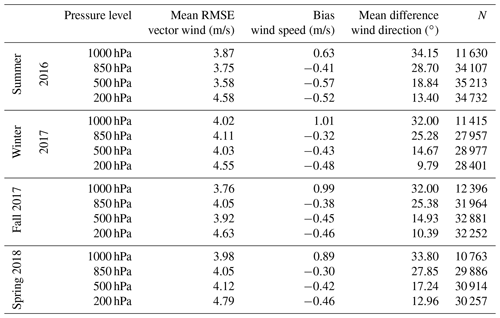Next, we use the ACT campaign airborne measurements to evaluate the MPAS-A CO2 simulation regarding its overall accuracy and its performance measured by three model evaluation metrics proposed by . To provide an objective reference, we also compare MPAS-A performance with two established CO2 model systems: WRF-Chem and CT2019 using the same set of airborne measurements. WRF-Chem is an online CTM based on the regional WRF model . WRF-Chem simulations have been carried out on a 27 km horizontal grid (Fig. S2) over North America as a part of the ACT campaign . The WRF-Chem simulations use ERA5 reanalysis for meteorological initial and lateral boundary conditions, CarbonTracker posterior mole fraction for CO2 initial and boundary conditions, and CarbonTracker posterior fluxes for surface CO2 fluxes. The WRF-Chem simulations use meteorological nudging and 120 h meteorological re-initialization to keep meteorological fields close to the reanalysis.

We use the ACT 5 s averaged CO2 measurement dataset , which has a horizontal resolution of approximately 500 m given the average aircraft velocity. MPAS-A simulated CO2 fields are sampled as described in the second paragraph of Sect. 3 to match the 5 s airborne data points. WRF-Chem simulated CO2 fields are also interpolated to match the ACT 5 s data point using the same approach as MPAS-A. CT2019 CO2 used for the evaluation is obtained from CarbonTracker ObsPack (v5.0) , which is the CT2019 posterior mole fraction interpolated to the ACT 5 s data points.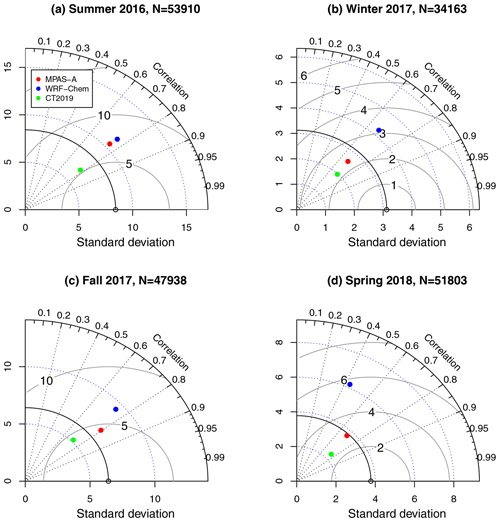Figure 8Taylor diagram for model evaluation using ACT airborne measurements in the boundary layer.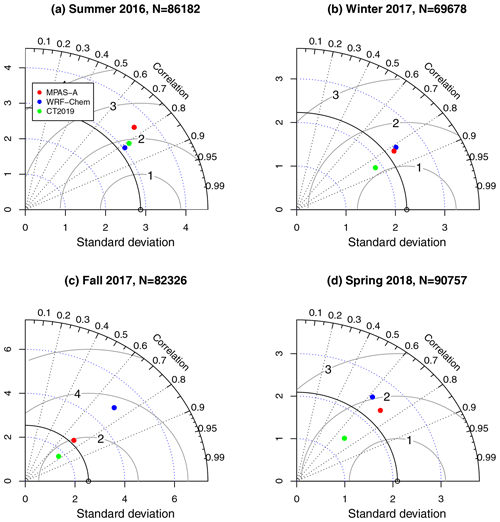Figure 9Taylor diagram for model evaluation using ACT airborne measurements in the free troposphere.

For each ACT flight day, CO2 measurements from the two aircraft are combined if both are available, and their corresponding modeled CO2 values from MPAS-A, WRF-Chem, and CT2019 are combined in the same way. With the four seasons combined, there are a total of 97 flight days , each one presented by an observation–model dataset consisting of observed CO2, modeled CO2 from the three models, along with the time, latitude, longitude, and altitude of each observation data point. Using the ACT maneuver flag dataset , we further divide each flight day's data into two groups: one for BL and another for FT. For each ACT campaign season, all the BL data–model pairs are combined for each of the three models for model comparison. Figure 8 shows the Taylor diagram of the model comparison in the BL for the four campaign seasons. N in the title of each figure is the number of model–data pairs used for plotting the diagram. Similarly, the model comparison in FT is summarized in the Taylor diagrams of Fig. 9. A comparison of Figs. 8 and 9 shows that all three models have higher accuracy (lower RMSE) in FT than BL, which could be attributed to the larger error in the weather forecast in BL than FT associated with the accuracy of PBL height in the model simulation. Figure 8 shows that in BL, MPAS-A has a higher RMSE and higher standard deviation than CT2019. MPAS-A has more accurate estimation of the observations' standard deviation than CT2019 in all but summer 2016. Compared with WRF-Chem, MPAS-A has a lower RMSE and more accurate estimation of the observations' standard deviation. Figure 9 shows that in FT, MPAS-A has higher RMSE than CT2019 in all four campaign seasons and but it has more accurate estimation of the observations' standard deviation than CT2019 in all but the summer 2016 season. Compared to WRF-Chem, MPAS-A has lower RMSE and more accurate estimation of observations' standard deviation in all but summer 2016.

### 3.4.1 Model representation of CO2 difference between warm and cold sectors

Through analyzing the ACT summer 2016 campaign data, identified three consistent features in the CO2 mole fraction and proposed using these features as transport model assessment metrics. The three features are the differences between the warm and cold sectors, the difference between the BL and FT, and the CO2 enhancement bands in the vicinity of frontal boundaries. Here and in the next two sections, we evaluate how MPAS-A simulated CO2 represents the three features.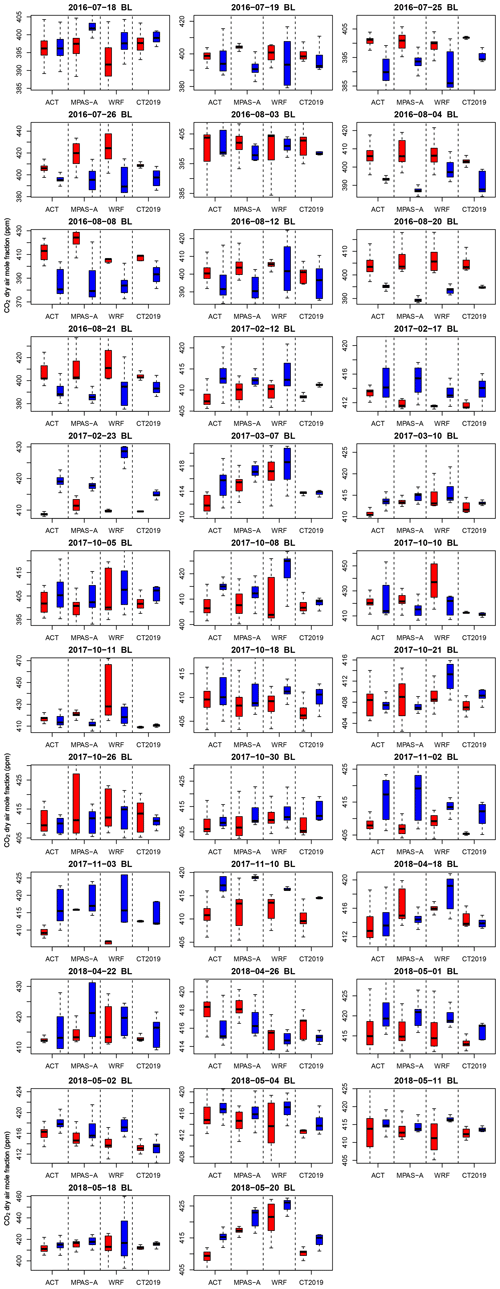Figure 10Box plots comparing mean boundary layer (BL) CO2 mole fraction of the warm sector (red color) and cold sector (blue color) for 15 frontal crossing flights from summer 2016 and winter 2017 ACT campaign seasons. The flight date of each plot is labeled in its title. Data are combined when both aircraft (C130 and B200) took measurements for a given day. Each subfigure is separated into four groups by the dotted lines: the first group is from ACT observations, the second is from the MPAS-A simulation, the third is from the WRF-Chem simulation, and the last is from CT2019. In each box plot, the bottom and top edges of the box represent the first (Q1) and third (Q3) quartiles, the horizontal line represents the median, the ends of the whiskers represent Q1  1.5 × IQR (interquartile range) and Q3 + 1.5 × IQR, respectively, where IQR = Q3  Q1.

Using the ACT maneuver flag dataset , we identified flights that crossed a weather front and their associated warm and cold sectors. The CO2 mole fraction statistics for the warm and cold sectors are calculated from the aircraft measurements and the modeled CO2 by MPAS-A, WRF-Chem, and CT2019, respectively. The results are shown in Fig. 10, which summarizes the statistics of CO2 mole fraction differences between the warm and cold sectors measured by 15 front-crossing flights: 10 from the summer 2016 season and 5 from the winter 2017 season. The figure confirms that the warm sector has a higher average CO2 mole fraction in the BL than the cold sector during summer 2016 as reported by . The figure also shows that the average CO2 mole fractions in the warm sectors are lower than those in the colder sectors in winter 2017, which is the opposite of what took place in summer 2016.

Table 11Comparison of mean CO2 dry-air mole fraction (ppm) in the boundary layer between the warm and the cold sectors. The table includes 10 frontal crossing flights from the summer 2016 season and 5 from the winter 2017 season. The column labeled “diff” is the mean value of the warm sector minus that of the cold sector.Table 11 lists the mean CO2 of the warm sector, cold sectors, and their difference as calculated from the ACT measurements, MPAS-A, WRF-Chem, and CT2019. The table shows that the MPAS-A simulations are similar to WRF-Chem, and both tend to have larger CO2 differences between the warm and cold sectors than CT2019. For instance, the 8 August 2016 case where the observed mean CO2 difference between the warm and cold sector is 26.9 ppm, MPAS-A and WRF simulations resulted in 36.9 and 21.2 ppm, respectively, while CT2019 resulted in a 15.3 ppm difference. The above evaluation indicates that the MPAS-A CO2 model is capable of well representing the observed CO2 difference between the warm and cold sectors, and its accuracy in this respect is comparable to WRF-Chem and CT2019.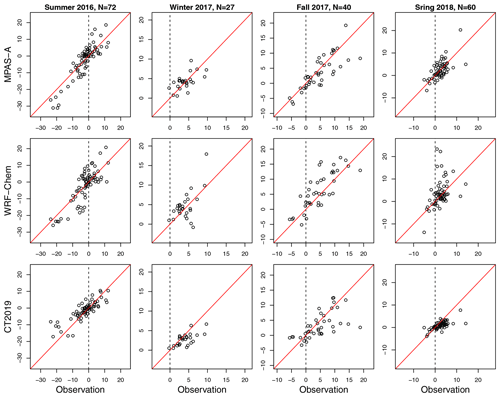Figure 11The difference of mean CO2 mole fraction between boundary layer (BL) and free troposphere (FT) ($\mathrm{\Delta }\left[{\mathrm{CO}}_{\mathrm{2}}\right]=\left[{\mathrm{CO}}_{\mathrm{2}}{\right]}_{\mathrm{BL}}-\left[{\mathrm{CO}}_{\mathrm{2}}{\right]}_{\mathrm{FT}}$) at vertical profiling flight legs. In each subplot, each open circle represents an individual vertical profiling flight leg, and its values on the x axis and y axis represent its Δ[CO2] value from the aircraft observations and the model simulation, respectively. Δ[CO2] values from MPAS-A simulations are in the first row, WRF-Chem the second row, and CT2019 the third. The four columns in the figure are for the four ACT campaign seasons. The number of vertical profiles in each season is labeled in the column title. The vertical dashed line marks where Δ[CO2]=0 based on the aircraft measurements.

Table 12Mean absolute error (MAE) of the simulated CO2 of MPAS-A, WRF-Chem, and CT2019 as validated using ACT campaign aircraft CO2 measurements. p values of paired t tests between MPAS-A and the other two models are included to provide a significance level for the model comparisons.### 3.4.2 Model representation of CO2 vertical difference

The second feature identified by is the vertical difference of CO2 mole fraction between the BL and FT. During the ACT campaign season, two research aircraft (B200 and C130) took many vertical profile measurements during take-off, landing, spiral-up and -down, and inline ascent and descent maneuvers (Pal2019). These profile observations characterize the vertical variation of the atmospheric CO2 mole fraction. From the vertical profile measurements taken during the summer 2016 season, calculated the mean CO2 mole fraction in the BL and FT, denoted as [CO2]BL and [CO2]FT, respectively. They further defined BL-to-FT CO2 difference as $\mathrm{\Delta }\left[{\mathrm{CO}}_{\mathrm{2}}\right]=\left[{\mathrm{CO}}_{\mathrm{2}}{\right]}_{\mathrm{BL}}-\left[{\mathrm{CO}}_{\mathrm{2}}{\right]}_{\mathrm{FT}}$. They found that Δ[CO2] tends to be positive in the warm sector and negative in the cold sector. In this section, we evaluate how well MPAS-A represents the BL-to-FT CO2 difference and compare its performance with WRF-Chem and CT2019.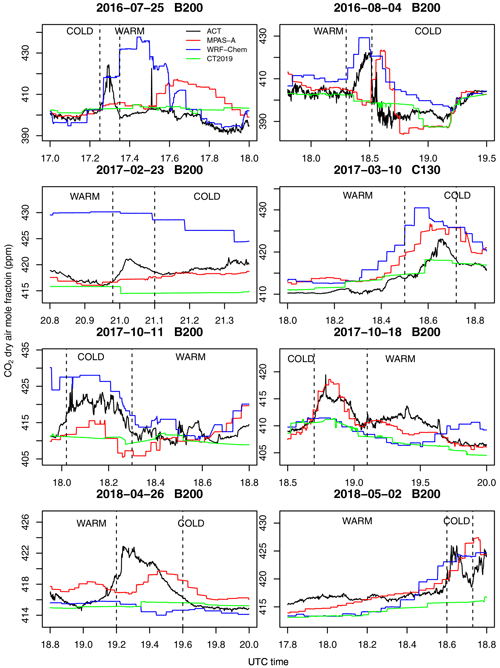Figure 12Comparison of CO2 mole fraction in frontal-crossing constant altitude flight segments in BL between ACT aircraft measurements and model simulations. Flight date and aircraft type are labeled in title for each flight leg. The x axis indicates UTC time, and the y axis shows the CO2 mole fraction (ppm). Aircraft measurements are in black, MPAS-A in red, WRF-Chem in blue, and CT2019 in green. In each figure, the pair of vertical dashed lines mark CO2 enhancement observed by the aircraft along a frontal boundary, and the warm and cold sectors associated with the frontal boundary are labeled as warm and cold, respectively.

Using the ACT maneuver flag dataset , we identified all vertical profiles taken during the four campaign seasons, from which we selected profiles that meet two criteria: (1) a vertical profile must include at least 20 5 s measurements in the BL and 20 measurements in FT; and (2) a vertical profile must extend at least 2 km in the vertical direction. These two criteria are used to ensure that the resulting [CO2]BL and [CO2]FT are statistically representative. A total of 199 qualified vertical profiles are identified from the four campaign seasons, including 72 from the summer 2016 season, 27 from winter 2017, 40 from fall 2017, and 60 from spring 2018. For each of the vertical profiles, Δ[CO2] is calculated for the aircraft CO2 measurements, and the simulated CO2 by MPAS-A, WRF-Chem, and CT2019. We compare Δ[CO2] from the models with that from the observations to assess how each model represents the observed BL-to-FT CO2 difference. Figure 11 shows the comparisons grouped by the campaign seasons. The figure indicates a clear distinction in Δ[CO2] between the summer 2016 and the other three seasons: there is a substantial number of both positive and negative Δ[CO2] in the summer 2016 season, but the vast majority of cases in the rest of the three campaign seasons have positive Δ[CO2]. The positive BL-to-FT CO2 differences from the winter 2017 season measurements could be at least partially attributable to the lack of CO2 drawdown during the non-growing season. In comparison, the fall 2017 and spring 2018 seasons have more mixed results probably because of their partial overlap with the growing season. For the summer 2016 season, vertical profiles with negative Δ[CO2] (lower mean CO2 in BL than FT) suggest photosynthesis during the growing season, but those with positive Δ[CO2] values are probably caused by the interaction between photosynthesis and frontal passage .

To compare the three models' accuracy in representing the BL-to-FT CO2 difference, we calculated the mean absolute error (MAE) for each model during each season, where $\mathrm{AE}=|\mathrm{\Delta }\left[{\mathrm{CO}}_{\mathrm{2}}{\right]}_{\mathrm{model}}-\mathrm{\Delta }\left[{\mathrm{CO}}_{\mathrm{2}}{\right]}_{\mathrm{obs}}|$ (the absolute difference in Δ[CO2] between a model and the ACT observations).

$\begin{array}{}\text{(21)}& \mathrm{MAE}=\frac{\mathrm{1}}{N}\sum _{i=\mathrm{1}}^{N}{\mathrm{AE}}_{i}\end{array}$

Table 12 summarize the MAE of the three models for each season. The table shows that MPAS-A has smaller MAE than CT2019 in fall 2017 (p=0.04) and a larger MAE in summer 2016 (p=0.06). The differences between the two models in the other two seasons are not significant (p≥0.23). Compared with WRF-Chem, MPAS-A has smaller MAEs in winter 2017 (p=0.09) and spring 2018 (p=0.01), while differences in the other two seasons are not significant (p≥0.11). In summary, the above model evaluation and comparison demonstrate that MPAS-A CO2 transport model is capable of representing the aircraft-observed CO2 difference between the BL and FT at least as accurately as WRF-Chem and CT2019.

### 3.4.3 Model representation of CO2 enhancement at frontal boundaries

The third feature identified by in the summer 2016 aircraft measurements is the bands of enhanced CO2 close to frontal boundaries in BL. They found these CO2 enhancement bands are typically about 100 km wide and speculated that it would require a 20 km horizontal resolution model to effectively represent the feature. In this section, we identify the frontal boundary CO2 enhancements in the four campaign seasons and examine how well they are represented by MPAS-A.

Using the same approach as , a total of 48 front-crossing constant-altitude flight segments are identified from the four seasons (15 from summer 2016, 5 from winter 2017, 17 from fall 2017, and 11 from fall 2018). To evaluate how well MPAS-A represents the frontal boundary CO2 enhancements and compare its performance with WRF-Chem and CT2019, CO2 mole fractions measured by the aircraft and simulated by the three models are plotted together for each of the identified front-crossing constant-altitude flight segments. Figure 12 includes eight of the front-crossing flight segments and the full set is included in Fig. S3 of the Supplement. For each flight segment in Fig. 12, the pair of vertical dashed lines mark CO2 enhancement observed by the aircraft along a frontal boundary. The warm and cold sectors associated with the frontal boundary in each flight are labeled as warm and cold, respectively. The figure indicates that frontal boundary CO2 enhancements can be identified in most but not all of the cases. For instance, there is no clearly identifiable CO2 enhancement in the B200 flights on 23 April 2018 (Fig. S3).

Figure 12 shows that MPAS-A has a varying degree of success in simulating the frontal boundary CO2 enhancements: it represents both the timing and the magnitude of the enhancements very well in some cases (4 August 2016 and 18 October 2017 by B200) but results in substantial errors in either the timing (25 July 2016, B200) or the magnitude (10 March 2017, C130) in other cases. The figure also shows that the MPAS-A simulated CO2 is more similar to WRF-Chem than CT2019: CT2019 tends to substantially underestimate the magnitude of CO2 enhancement, while MPAS-A and WRF-Chem tend to overestimate it.Figure 13MPAS-A simulated equivalent potential temperature (θe, a) and CO2 mole fraction (b) at 18:00 UTC on 4 August 2016. Both figures are plotted at the sixth MPAS-A vertical level, which is about 400 m above the ground.

Figure 13 shows the MPAS-A simulated equivalent potential temperature (θe) and CO2 mole fraction at 18:00 UTC on 4 August 2016. The sharp boundary in θe indicates a surface cold front extending from southern Colorado northeastward to Wisconsin. Abrupt horizontal wind direction changes shown in Fig. S4 of the Supplement also indicate the cold front and its southeastward movement. Meteorological measurements taken during the flight (not shown) also confirm the cold front passage. The B200 research aircraft crossed the cold front from southeast to northwest at about 400–500 m above the ground between 17:15 and 19:15 UTC, and its flight track and timing are marked in Fig. 13. The aircraft measurements show an approximately 20 ppm enhancement along the front boundary, which can be clearly identified in the MPAS-A simulated CO2 mole fraction (lower panel of Fig. 13).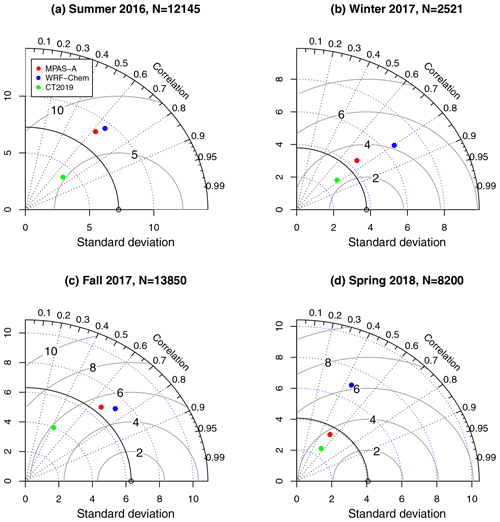Figure 14Taylor diagram for model evaluation using ACT airborne measurements from front-crossing flights. For each of the four ACT campaign seasons, observation–model data pairs from all front-crossing flights are combined. N is the number of data pairs used for creating the diagram.

Figure 14 compares the three models in their representation of the frontal boundary CO2 variation. The figure shows that except for summer 2016, MPAS-A has a similar level of RMSE to CT2019, and it has a more accurate estimation of the observations' standard deviation. As horizontal resolution impacts a model's ability to represent small-scale spatial variability , the coarser resolution of CT2019 (1× 1 over North America) is likely the primary cause of its underestimation of the frontal boundary CO2 variability. MPAS-A has lower RMSE than WRF-Chem in winter 2017 and spring 2018, and it has a similar RMSE to WRF-Chem in the other two seasons. In all but summer 2016, MPAS-A has a more accurate estimation of the observations' standard deviation than WRF-Chem.

4 Summary

We implemented the CO2 atmospheric transport processes, including advection, vertical mixing, and convective transport, in the global variable-resolution MPAS-A model. After the model development details were presented, simulation experiments designed for model evaluation were described. Two sets of simulations over a 60–15 km variable-resolution global domain were conducted for a model accuracy evaluation using an extensive aircraft measurements over the eastern United States and near-surface hourly measurements from surface and tower stations distributed across the globe. Meteorological initial conditions for these simulations are from the ERA-Interim analysis , and CO2 initial conditions and fluxes are from CT2019 posterior mole fraction and flux products . To keep model meteorological fields close to the analysis, meteorology re-initialization is applied at 24 h intervals throughout the simulation periods. Global CO2 mass conservation property is assessed by a 1-year continuous simulation without meteorology re-initialization and fluxes, and the results show that MPAS-A is capable of maintaining total dry-air mass conservation to the limit of machine precision. During the 1-year simulation period, the total CO2 mass change is about 10−5 of its initial value. The larger variation of CO2 mass than the dry air is due to the complex and strong spatial gradient caused by the surface CO2 fluxes. Another 1-year simulation with meteorology re-initialization indicates that changes in dry-air density during the re-initialization causes changes in global total CO2 mass, and a scaling method applied after each re-initialization is able to reduce the change from $\sim {\mathrm{10}}^{-\mathrm{4}}$ to $\sim {\mathrm{10}}^{-\mathrm{6}}$ of the global CO2 mass.

The horizontal wind fields of the 60–15 km variable-resolution MPAS-A simulation are evaluated at four pressure levels at 457 radiosonde stations. Furthermore, a comparison with an additional 60 km uniform-resolution MPAS-A simulation shows that the accuracy of the horizontal wind fields is substantially higher at the 15 km cells. The accuracy of MPAS-A CO2 transport is evaluated first at the global scale and then at the regional scale. At the global scale, MPAS-A simulation is evaluated using CT2019, near-surface hourly CO2 measurements from 50 stations and XCO2 measurements from 18 TCCON stations. The resulting statistics are compared with the ECMWF IFS 9 and 80 km resolution simulations over the same period conducted by . The comparison indicates that RMSE of the MPAS-A simulation is similar to that of the 80 km IFS simulation but larger than that of the 9 km IFS simulation.

At the regional scale, a MPAS-A simulation extending from 1 January 2016 to 1 June 2018 is evaluated using the extensive high-resolution aircraft measurements from four ACT campaign seasons. Compared with a 27 km resolution WRF-Chem simulation and CT2019 posterior CO2 mole fraction, MPAS-A simulated CO2 achieves a comparable level of accuracy (as measured by RMSE). Further evaluation using three metrics proposed by shows that the MPAS-A simulation is capable of representing the observed CO2 features as accurately as the WRF-Chem simulation and CT2019.

The model evaluations using the airborne and near-surface measurements indicate that the newly developed MPAS-A CO2 transport model is capable of achieving a comparable level of accuracy with the more established CO2 modeling systems, including the WRF-Chem regional model system, the CT2019 operational assimilation system, and the lower-resolution (80 km) simulation of the ECMWF IFS global CO2 modeling system. Although further improvements are expected, the MPAS-A CO2 transport model has the potential to contribute to improving our knowledge of atmospheric CO2 transport and fluxes.

Code and data availability

Source code for the MPAS-A CO2 transport model v6.3 can be retrieved at https://doi.org/10.5281/zenodo.3976320 (Zheng2020). Source code for WRF-Chem v3.6 used in the manuscript can be obtained from the NCAR website at http://www2.mmm.ucar.edu/wrf/users/download/get_source.html (last access: 25 May 2021). Source code for IFS is only available subject to a license agreement with ECMWF. ECMWF member-state weather services and their approved partners will have access granted. The IFS source code without modules for assimilation and chemistry can be obtained for educational and academic purposes as part of the OpenIFS release (https://software.ecmwf.int/wiki/display/OIFS/OpenIFS+Home, last access: 25 May 2021). ACT-America in situ airborne CO2 measurement data can be obtained from https://doi.org/10.3334/ORNLDAAC/1593 . Surface- and tower-based CO2 measurement data from ObsPack GLOBALVIEWplus v5.0 can be obtained from the NOAA website: https://esrl.noaa.gov/gmd/ccgg/obspack/data.php (last access: 25 May 2021). TCCON data can be obtained from https://tccondata.org/ (last access: 25 May 2021). CarbonTracker CO2 flux and posterior mixing ratio data can be obtained from the NOAA website: https://www.esrl.noaa.gov/gmd/ccgg/carbontracker/download.php (last access: 25 May 2021).

Supplement

Author contributions

TZ implemented the CO2 transport processes in MPAS-A v6.3. SF conducted the WRF-Chem 27 km simulations. TZ, SF, KJD, and SP designed model evaluation using ACT-America aircraft measurements. TZ and JAM designed the model evaluation using continuous in situ tower measurements. TZ, SF, KJD, and SP analyzed the model representation of distinct CO2 spatial features observed by the aircraft measurements. All authors contributed to writing and commenting on the paper.

Competing interests

The authors declare that they have no conflict of interest.

Acknowledgements

We thank the MPAS-A development team for making their code available to the public. We thank the NOAA CarbonTracker team for providing the CT2019 flux and mole fraction data. We thank ECMWF for the ERA-Interim analysis data; we thank the ObsPack data providers for the in situ continuous CO2 measurement data. We thank the TCCON PIs for providing the dataset. Sha Feng and Kenneth J. Davis were supported by the Atmospheric Carbon and Transport (ACT) – America Earth Venture Suborbital 2 project funded by NASA's Earth Science Division (grant no. NNX15AG76G to Penn State). Sandip Pal was supported by NASA (grant no. 80NSSC19K0730) and a Texas Tech University start-up research grant. This work was supported in part through computational resources and services provided by the Institute for Cyber-Enabled Research at Michigan State University. This is contribution 154 of the Central Michigan University Institute for Great Lakes Research. We acknowledge partial support for the publication fee by the Central Michigan University FRCE fund. We thank the two anonymous reviewers for their thorough and constructive comments which helped to improve this paper.

Financial support

This research has been supported by the National Aeronautics and Space Administration (NASA) Earth Science Division, USA (grant no. NNX15AG76G to Pennsylvania State University, USA).

Review statement

This paper was edited by Juan Antonio Añel and reviewed by two anonymous referees.

References

Agustí-Panareda, A., Massart, S., Chevallier, F., Boussetta, S., Balsamo, G., Beljaars, A., Ciais, P., Deutscher, N. M., Engelen, R., Jones, L., Kivi, R., Paris, J.-D., Peuch, V.-H., Sherlock, V., Vermeulen, A. T., Wennberg, P. O., and Wunch, D.: Forecasting global atmospheric CO2, Atmos. Chem. Phys., 14, 11959–11983, https://doi.org/10.5194/acp-14-11959-2014, 2014. a, b, c

Agusti-Panareda, A., Diamantakis, M., Bayona, V., Klappenbach, F., and Butz, A.: Improving the inter-hemispheric gradient of total column atmospheric CO2 and CH4 in simulations with the ECMWF semi-Lagrangian atmospheric global model, Geosci. Model Dev., 10, 1–18, https://doi.org/10.5194/gmd-10-1-2017, 2017. a

Agustí-Panareda, A., Diamantakis, M., Massart, S., Chevallier, F., Muñoz-Sabater, J., Barré, J., Curcoll, R., Engelen, R., Langerock, B., Law, R. M., Loh, Z., Morguí, J. A., Parrington, M., Peuch, V.-H., Ramonet, M., Roehl, C., Vermeulen, A. T., Warneke, T., and Wunch, D.: Modelling CO2 weather – why horizontal resolution matters, Atmos. Chem. Phys., 19, 7347–7376, https://doi.org/10.5194/acp-19-7347-2019, 2019. a, b, c, d, e, f, g, h, i, j, k, l, m, n, o, p, q

Andrews, A. E., Kofler, J. D., Trudeau, M. E., Williams, J. C., Neff, D. H., Masarie, K. A., Chao, D. Y., Kitzis, D. R., Novelli, P. C., Zhao, C. L., Dlugokencky, E. J., Lang, P. M., Crotwell, M. J., Fischer, M. L., Parker, M. J., Lee, J. T., Baumann, D. D., Desai, A. R., Stanier, C. O., De Wekker, S. F. J., Wolfe, D. E., Munger, J. W., and Tans, P. P.: CO2, CO, and CH4 measurements from tall towers in the NOAA Earth System Research Laboratory's Global Greenhouse Gas Reference Network: instrumentation, uncertainty analysis, and recommendations for future high-accuracy greenhouse gas monitoring efforts, Atmos. Meas. Tech., 7, 647–687, https://doi.org/10.5194/amt-7-647-2014, 2014. a, b, c, d, e, f, g

Baker, D. F., Doney, S. C., and Schimel, D. S.: Variational data assimilation for atmospheric CO2, Tellus B, 58, 359–365, 2006. a

Blumenstock, T., Hase, F., Schneider, M., García, O. E., and Sepúlveda, E.: TCCON data from Izana (ES), Release GGG2014.R1, https://doi.org/10.14291/TCCON.GGG2014.IZANA01.R1, 2017. a

Borge, R., Alexandrov, V., del Vas, J. J., Lumbreras, J., and Rodriguez, E.: A comprehensive sensitivity analysis of the WRF model for air quality applications over the Iberian Peninsula, Atmos. Environ., 42, 8560–8574, https://doi.org/10.1016/j.atmosenv.2008.08.032, 2008. a

Brunke, E., Labuschagne, C., Parker, B., Scheel, H., and Whittlestone, S.: Baseline air mass selection at Cape Point, South Africa: application of Rn-222 and other filter criteria to CO2, Atmos. Environ., 38, 5693–5702, https://doi.org/10.1016/j.atmosenv.2004.04.024, 2004. a

Chen, F. and Dudhia, J.: Coupling an advanced land surface-hydrology model with the Penn State-NCAR MM5 modeling system. Part I: Model implementation and sensitivity, Mon. Weather Rev., 129, 569–585, https://doi.org/10.1175/1520-0493(2001)129<0569:CAALSH>2.0.CO;2, 2001. a, b

Conway, T. J. and Thoning, K. W.: Short-term variations of atmospheric carbon dioxide at the South Pole, Anarctic J., 25, 236–238, 1990. a

Davies, T.: Lateral boundary conditions for limited area models, Q. J. Roy. Meteor. Soc., 140, 185–196, https://doi.org/10.1002/qj.2127, 2014. a

Davis, K., Baier, B., Z., B., Bowman, K., Boyer, A., and Browell, E.: Atmospheric Carbon and Transport (ACT) – America: A multi‐year airborne mission to study fluxes and transport of CO2 and CH4 across the eastern United States, American Geophysical Union Fall Meeting, San Francisco, CA, USA, 2018a. a

Davis, K. J., Obland, M. D., Lin, B., Lauvaux, T., O'Dell, C., Meadows, B., Browell, E. V., DiGangi, J. P., Sweeney, C., McGill, M. J., Barrick, J. D., Nehrir, A. R., Yang, M. M., Bennett, J. R., Baier, B. C., Roiger, A., Pal, S., Gerken, T., Fried, A., Feng, S., Shrestha, R., Shook, M. A., Chen, G., Campbell, L. J., Barkley, Z. R., and Pauly, R. M.: ACT–America: L3 Merged In Situ Atmospheric Trace Gases and Flask Data, Eastern USA [Data set], ORNL DAAC, Oak Ridge, Tennessee, USA, https://doi.org/10.3334/ORNLDAAC/1593, 2018b. a, b

Dee, D. P., Uppala, S. M., Simmons, A. J., Berrisford, P., Poli, P., Kobayashi, S., Andrae, U., Balmaseda, M. A., Balsamo, G., Bauer, P., Bechtold, P., Beljaars, A. C. M., van de Berg, L., Bidlot, J., Bormann, N., Delsol, C., Dragani, R., Fuentes, M., Geer, A. J., Haimberger, L., Healy, S. B., Hersbach, H., Holm, E. V., Isaksen, L., Kallberg, P., Koehler, M., Matricardi, M., McNally, A. P., Monge-Sanz, B. M., Morcrette, J. J., Park, B. K., Peubey, C., de Rosnay, P., Tavolato, C., Thepaut, J. N., and Vitart, F.: The ERA-Interim reanalysis: configuration and performance of the data assimilation system, Q. J. Roy. Meteor. Soc.,, 137, 553–597, https://doi.org/10.1002/qj.828, 2011. a, b

De Mazière, M., Sha, M. K., Desmet, F., Hermans, C., Scolas, F., Kumps, N., Metzger, J.-M., Duflot, V., and Cammas, J.-P.: TCCON data from Réunion Island (RE), Release GGG2014.R0, https://doi.org/10.14291/TCCON.GGG2014.REUNION01.R0/ 1149288, 2014. a

Deutscher, N. M., Notholt, J., Messerschmidt, J., Weinzierl, C., Warneke, T., Petri, C., and Grupe, P.: TCCON data from Bialystok (PL), Release GGG2014.R1, https://doi.org/10.14291/TCCON.GGG2014.BIALYSTOK01.R1/ 1183984, 2015. a

Diamantakis, M. and Flemming, J.: Global mass fixer algorithms for conservative tracer transport in the ECMWF model, Geosci. Model Dev., 7, 965–979, https://doi.org/10.5194/gmd-7-965-2014, 2014. a, b

Díaz-Isaac, L. I., Lauvaux, T., and Davis, K. J.: Impact of physical parameterizations and initial conditions on simulated atmospheric transport and CO2 mole fractions in the US Midwest, Atmos. Chem. Phys., 18, 14813–14835, https://doi.org/10.5194/acp-18-14813-2018, 2018. a

Díaz-Isaac, L. I., Lauvaux, T., Bocquet, M., and Davis, K. J.: Calibration of a multi-physics ensemble for estimating the uncertainty of a greenhouse gas atmospheric transport model, Atmos. Chem. Phys., 19, 5695–5718, https://doi.org/10.5194/acp-19-5695-2019, 2019. a

Feist, D. G., Arnold, S. G., John, N., and Geibel, M. C.: TCCON data from Ascension Island, Saint Helena, Ascension and Tristan da Cunha, Release GGG2014R0, TCCON data archive, hosted by the Carbon Dioxide Information Analysis Center, Oak Ridge National Laboratory, Oak Ridge, Tennessee, USA, https://doi.org/10.14291/tccon.ggg2014.ascension01.R0/1149285, 2014. a

Feng, S., Lauvaux, T., Barkley, Z. R., Butler, M. B., Deng, A., Gaudet, B., and Davis, K. J.: Full WRF-Chem output in support of the NASA Atmospheric Carbon and Transport (ACT)-America project (7/1/2016 – 7/31/2019). The Pennsylvania State University Data Commons, University Park, Pennsylvania, USA, https://doi.org/10.26208/49kd-b637, 2020. a

Feng, S., Lauvaux, T., Newman, S., Rao, P., Ahmadov, R., Deng, A., Díaz-Isaac, L. I., Duren, R. M., Fischer, M. L., Gerbig, C., Gurney, K. R., Huang, J., Jeong, S., Li, Z., Miller, C. E., O'Keeffe, D., Patarasuk, R., Sander, S. P., Song, Y., Wong, K. W., and Yung, Y. L.: Los Angeles megacity: a high-resolution land–atmosphere modelling system for urban CO2 emissions, Atmos. Chem. Phys., 16, 9019–9045, https://doi.org/10.5194/acp-16-9019-2016, 2016. a, b

Feng, S., Lauvaux, T., Davis, K. J., Keller, K., Zhou, Y., Williams, C., Schuh, A. E., Liu, J., and Baker, I.: Seasonal Characteristics of Model Uncertainties From Biogenic Fluxes, Transport, and Large-Scale Boundary Inflow in Atmospheric CO2 Simulations Over North America, J. Geophys. Res.-Atmos., 124, 14325–14346, https://doi.org/10.1029/2019JD031165, 2019. a, b

Francey, R. J., Steele, L. P., Spencer, D. A., Langenfelds, R. L., Law, R. M., Krummel, P. B., Fraser, P. J., Etheridge, D. M., Derek, N., Coram, S. A., Cooper, L. N., Allison, C. E., Porter, L., and Baly, S.: The CSIRO (Australia) measurement of greenhouse gases in the global atmosphere, report of the 11th WMO/IAEA Meeting of Experts on Carbon Dioxide Concentration and Related Tracer Measurement Techniques, Tokyo, Japan, September 2001, edited by: Toru, S. and Kazuto, S., World Meteorological Organization Global Atmosphere Watch, Geneva, Switzerland, 2003. a

Fritsch, J. M. and Chappell, C. F.: Numerical prediction of convectively driven mesoscale pressure systems. Part I: convective parameterization, J. Atmos. Sci., 37, 1722–1733, https://doi.org/10.1175/1520-0469(1980)037<1722:NPOCDM>2.0.CO;2, 1980. a

Gaudry, A., Monfray, P., Polian, G., Bonsang, G., Ardouin, B., Jegou, A., and Lambert, G.: Nonseasonnal variations of atmospheric CO2 concentrations at Amsterdam Island, Tellus B, 43, 136–143, https://doi.org/10.1034/j.1600-0889.1991.00008.x, 1991. a

Gerbig, C., Körner, S., and Lin, J. C.: Vertical mixing in atmospheric tracer transport models: error characterization and propagation, Atmos. Chem. Phys., 8, 591–602, https://doi.org/10.5194/acp-8-591-2008, 2008. a

Gerbig, C., Dolman, A. J., and Heimann, M.: On observational and modelling strategies targeted at regional carbon exchange over continents, Biogeosciences, 6, 1949–1959, https://doi.org/10.5194/bg-6-1949-2009, 2009. a

Gockede, M., Turner, D. P., Michalak, A. M., Vickers, D., and Law, B. E.: Sensitivity of a subregional scale atmospheric inverse CO2 modeling framework to boundary conditions, J. Geophys. Res., 115, D24112, https://doi.org/10.1029/2010JD014443, 2010. a

Golaz, J.-C., Caldwell, P. M., Van Roekel, L. P., Petersen, M. R., Tang, Q., Wolfe, J. D., Abeshu, G., Anantharaj, V., Asay-Davis, X. S., Bader, D. C., Baldwin, S. A., Bisht, G., Bogenschutz, P. A., Branstetter, M., Brunke, M. A., Brus, S. R., Burrows, S. M., Cameron-Smith, P. J., Donahue, A. S., Deakin, M., Easter, R. C., Evans, K. J., Feng, Y., Flanner, M., Foucar, J. G., Fyke, J. G., Griffin, B. M., Hannay, 15 C., Harrop, B. E., Hoffman, M. J., Hunke, E. C., Jacob, R. L., Jacobsen, D. W., Jeffery, N., Jones, P. W., Keen, N. D., Klein, S. A., Larson, V. E., Leung, L. R., Li, H.-Y., Lin, W., Lipscomb, W. H., Ma, P.-L., Mahajan, S., Maltrud, M. E., Mametjanov, A., McClean, J. L., McCoy, R. B., Neale, R. B., Price, S. F., Qian, Y., Rasch, P. J., Eyre, J. E. J. R., Riley, W. J., Ringler, T. D., Roberts, A. F., Roesler, E. L., Salinger, A. G., Shaheen, Z., Shi, X., Singh, B., Tang, J., Taylor, M. A., Thornton, P. E., Turner, A. K., Veneziani, M., Wan, H., Wang, H., Wang, S., Williams, D. N., Wolfram, P. J., Worley, P. H., Xie, S., Yang, Y., Yoon, J.-H., Zelinka, M. D., Zender, C. S., Zeng, X., Zhang, C., Zhang, K., Zhang, Y., Zheng, X., Zhou, T., and Zhu, Q.: The DOE E3SM Coupled Model Version 1: Overview and Evaluation at Standard Resolution, J. Adv. Model. Earth Sy., 11, 2089–2129, https://doi.org/10.1029/2018MS001603, 2019. a

Gomez-Pelaez, A. J. and Ramos, R.: Improvements in the Carbon Dioxide and Methane Continuous Measurement Programs at Izana Global GAW Station (Spain) during 2007–2009, in: GAW report (No. 194) of the 15th WMO/IAEA Meeting of Experts on Carbon Dioxide, Other Greenhouse Gases, and Related Tracer Measurement Techniques, Jena, Germany; 7–10 September 2009, edited by: Brand, W. A., World Meteorological Organization, TD No. 1553, 2005. a

Grell, G., Freitas, S. R., Stuefer, M., and Fast, J.: Inclusion of biomass burning in WRF-Chem: impact of wildfires on weather forecasts, Atmos. Chem. Phys., 11, 5289–5303, https://doi.org/10.5194/acp-11-5289-2011, 2011. a

Griffith, D. W., Deutscher, N. M., Velazco, V. A., Wennberg, P. O., Yavin, Y., Keppel-Aleks, G., Washenfelder, R. A., Toon, G. C., Blavier, J.-F., Paton-Walsh, C., Jones, N. B., Kettlewell, G. C., Connor, B. J., Macatangay, R. C., Roehl, C., Ryczek, M., Glowacki, J., Culgan, T., and Bryant, G. W.: TCCON data from Darwin (AU), Release GGG2014.R0, https://doi.org/10.14291/TCCON.GGG2014.DARWIN01.R0/ 1149290, 2014a. a

Griffith, D. W., Velazco, V. A., Deutscher, N. M., Paton-Walsh, C., Jones, N. B., Wilson, S. R., Macatangay, R. C., Kettlewell, G. C., Buchholz, R. R., and Riggenbach, M. O.: TCCON data from Wollongong (AU), Release GGG2014.R0, https://doi.org/10.14291/TCCON.GGG2014.WOLLONGONG01.R0/ 1149291, 2014b. a

Halter, B., Harris, J., and Conway, T.: Component signals in the record of atmospheric carbon dioxide concentration at American Samoa, J. Geophys. Res.-Atmos., 93, 15914–15918, https://doi.org/10.1029/JD093iD12p15914, 1988. a

Hase, F., Blumenstock, T., Dohe, S., Groß, J., and Kiel, M.: TCCON data from Karlsruhe (DE), Release GGG2014.R1, https://doi.org/10.14291/TCCON.GGG2014.KARLSRUHE01. R1/1182416, 2015. a

Haszpra, L., Barcza, Z., Bakwin, P., Berger, B., Davis, K., and Weidinger, T.: Measuring system for the long-term monitoring of biosphere/atmosphere exchange of carbon dioxide, J. Geophys. Res.-Atmos., 106, 3057–3069, https://doi.org/10.1029/2000JD900600, 2001. a

Hatakka, J., Aalto, T., Aaltonen, V., Aurela, M., Hakola, H., Komppula, M., Laurila, T., Lihavainen, H., Paatero, J., Salminen, K., and Viisanen, Y.: Overview of the atmospheric research activities and results at Pallas GAW station, Boreal Environ. Res., 8, 365–383, 2003. a

Hersbach, H., Bell, B., Berrisford, P., Hirahara, S., Horanyi, A., Munoz-Sabater, J., Nicolas, J., Peubey, C., Radu, R., Schepers, D., Simmons, A., Soci, C., Abdalla, S., Abellan, X., Balsamo, G., Bechtold, P., Biavati, G., Bidlot, J., Bonavita, M., De Chiara, G., Dahlgren, P., Dee, D., Diamantakis, M., Dragani, R., Flemming, J., Forbes, R., Fuentes, M., Geer, A., Haimberger, L., Healy, S., Hogan, R. J., Holm, E., Janiskova, M., Keeley, S., Laloyaux, P., Lopez, P., Lupu, C., Radnoti, G., de Rosnay, P., Rozum, I., Vamborg, F., Villaume, S., and Thepaut, J.-N.: The ERA5 global reanalysis, Q. J. Roy. Meteor. Soc., 146, 1999–2049, https://doi.org/10.1002/qj.3803, 2020. a

Hong, S., Dudhia, J., and Chen, S.: A revised approach to ice microphysical processes for the bulk parameterization of clouds and precipitation, Mon. Weather Rev., 132, 103–120, https://doi.org/10.1175/1520-0493(2004)132<0103:ARATIM>2.0.CO;2, 2004. a

Hong, S.-Y. and Lim, J.: The WRF single-moment microphysics scheme (WSM6), Journal of the Korean Meteorological Society, 42, 129–151, 2006. a, b

Hong, S.-Y., Noh, Y., and Dudhia, J.: A new vertical diffusion package with an explicit treatment of entrainment processes, Mon. Weather Rev., 134, 2318–2341, https://doi.org/10.1175/MWR3199.1, 2006. a, b, c, d, e, f, g, h

Hu, L., Andrews, A. E., Thoning, K. W., Sweeney, C., Miller, J. B., Michalak, A. M., Dlugokencky, E., Tans, P. P., Shiga, Y. P., Mountain, M., Nehrkorn, T., Montzka, S. A., McKain, K., Kofler, J., Trudeau, M., Michel, S. E., Biraud, S. C., Fischer, M. L., Worthy, D. E. J., Vaughn, B. H., White, J. W. C., Yadav, V., Basu, S., and van der Velde, I. R.: Enhanced North American carbon uptake associated with El Niño, Sci. Adv., 5, eaaw0076, https://doi.org/10.1126/sciadv.aaw0076, 2019. a

Hu, X.-M., Nielsen-Gammon, J. W., and Zhang, F.: Evaluation of Three Planetary Boundary Layer Schemes in the WRF Model, J. Appl. Meteorol. Climatol., 49, 1831–1844, 2010. a

Iacono, M. J., Delamere, J. S., Mlawer, E. J., Shephard, M. W., Clough, S. A., and Collins, W. D.: Radiative forcing by long-lived greenhouse gases: Calculations with the AER radiative transfer models, J. Geophys. Res.-Atmos., 113, D13103, https://doi.org/10.1029/2008JD009944, 2008. a, b, c

Iraci, L. T., Podolske, J. R., Hillyard, P. W., Roehl, C., Wennberg, P. O., Blavier, J.-F., Landeros, J., Allen, N., Wunch, D., Zavaleta, J., Quigley, E., Osterman, G. B., Albertson, R., Dunwoody, K., and Boyden, H.: TCCON data from Edwards (US), Release GGG2014.R1, https://doi.org/10.14291/TCCON.GGG2014.EDWARDS01.R1/ 1255068, 2016. a

Jacobson, A. R., Fletcher, S. E. M., Gruber, N., Sarmiento, J. L., and Gloor, M.: A joint atmosphere-ocean inversion for surface fluxes of carbon dioxide: 1. Methods and global-scale fluxes, Global Biogeochem. Cycles, 21, GB1020, https://doi.org/10.1029/2006GB002703, 2007. a

Jacobson, A. R., Schuldt, K. N., Miller, J. B., Oda, T., Tans, P., Arlyn Andrews, Mund, J., Ott, L., Collatz, G. J., Aalto, T., Afshar, S., Aikin, K., Aoki, S., Apadula, F., Baier, B., Bergamaschi, P., Beyersdorf, A., Biraud, S. C., Bollenbacher, A., Bowling, D., Brailsford, G., Abshire, J. B., Chen, G., Huilin Chen, Lukasz Chmura, Sites Climadat, Colomb, A., Conil, S., Cox, A., Cristofanelli, P., Cuevas, E., Curcoll, R., Sloop, C. D., Davis, K., Wekker, S. D., Delmotte, M., DiGangi, J. P., Dlugokencky, E., Ehleringer, J., Elkins, J. W., Emmenegger, L., Fischer, M. L., Forster, G., Frumau, A., Galkowski, M., Gatti, L. V., Gloor, E., Griffis, T., Hammer, S., Haszpra, L., Hatakka, J., Heliasz, M., Hensen, A., Hermanssen, O., Hintsa, E., Holst, J., Jaffe, D., Karion, A., Kawa, S. R., Keeling, R., Keronen, P., Kolari, P., Kominkova, K., Kort, E., Krummel, P., Kubistin, D., Labuschagne, C., Langenfelds, R., Laurent, O., Laurila, T., Lauvaux, T., Law, B., Lee, J., Lehner, I., Leuenberger, M., Levin, I., Levula, J., Lin, J., Lindauer, M., Loh, Z., Lopez, M., Myhre, C. L., Machida, T., Mammarella, I., Manca, G., Manning, A., Manning, A., Marek, M. V., Marklund, P., Martin, M. Y., Matsueda, H., McKain, K., Meijer, H., Meinhardt, F., Miles, N., Miller, C. E., Mölder, M., Montzka, S., Moore, F., Josep-Anton Morgui, Morimoto, S., Munger, B., Jaroslaw Necki, Newman, S., Nichol, S., Niwa, Y., O'Doherty, S., Mikaell Ottosson-Löfvenius, Paplawsky, B., Peischl, J., Peltola, O., Jean-Marc Pichon, Piper, S., Plass-Dölmer, C., Ramonet, M., Reyes-Sanchez, E., Richardson, S., Riris, H., Ryerson, T., Saito, K., Sargent, M., Sasakawa, M., Sawa, Y., Say, D., Scheeren, B., Schmidt, M., Schmidt, A., Schumacher, M., Shepson, P., Shook, M., Stanley, K., Steinbacher, M., Stephens, B., Sweeney, C., Thoning, K., Torn, M., Turnbull, J., Tørseth, K., Bulk, P. V. D., Laan-Luijkx, I. T. V. D., Dinther, D. V., Vermeulen, A., Viner, B., Vitkova, G., Walker, S., Weyrauch, D., Wofsy, S., Worthy, D., Dickon Young, and Miroslaw Zimnoch: CarbonTracker CT2019, https://doi.org/10.25925/39M3-6069, 2020. a, b, c, d, e, f, g, h, i

Kain, J. S.: The Kain–Fritsch Convective Parameterization: An Update, J. Appl. Meteorol., 43, 170–181, https://doi.org/10.1175/1520-0450(2004)043<0170:TKCPAU>2.0.CO;2, 2004. a, b, c, d, e, f

Kain, J. S. and Fritsch, J. M.: A one-dimensional entraining detraining plume model and its application in convective parameterization, J. Atmos. Sci., 47, 2784–2802, https://doi.org/10.1175/1520-0469(1990)047<2784:AODEPM>2.0.CO;2, 1990. a

Kawakami, S., Ohyama, H., Arai, K., Okumura, H., Taura, C., Fukamachi, T., and Sakashita, M.: TCCON data from Saga (JP), Release GGG2014.R0, https://doi.org/10.14291/TCCON.GGG2014.SAGA01.R0/1149 283, 2014. a

Kivi, R., Heikkinen, P., and Kyro: TCCON data from Sodankyla, Finland, Release GGG2014R0., TCCON data archive, hosted by the Carbon Dioxide Information Analysis Center, Oak Ridge National Laboratory, Oak Ridge, Tennessee, USA, https://doi.org/10.14291/tccon.ggg2014.sodankyla01.R0/1149280, 2014. a

Kretschmer, R., Gerbig, C., Karstens, U., and Koch, F.-T.: Error characterization of CO2 vertical mixing in the atmospheric transport model WRF-VPRM, Atmos. Chem. Phys., 12, 2441–2458, https://doi.org/10.5194/acp-12-2441-2012, 2012. a

Krol, M., Houweling, S., Bregman, B., van den Broek, M., Segers, A., van Velthoven, P., Peters, W., Dentener, F., and Bergamaschi, P.: The two-way nested global chemistry-transport zoom model TM5: algorithm and applications, Atmos. Chem. Phys., 5, 417–432, https://doi.org/10.5194/acp-5-417-2005, 2005. a, b

Lauvaux, T. and Davis, K. J.: Planetary boundary layer errors in mesoscale inversions of column-integrated CO2 measurements, J. Geophys. Res.-Atmos., 119, 490–508, https://doi.org/10.1002/2013JD020175, 2014. a

Lauvaux, T., Schuh, A. E., Uliasz, M., Richardson, S., Miles, N., Andrews, A. E., Sweeney, C., Diaz, L. I., Martins, D., Shepson, P. B., and Davis, K. J.: Constraining the CO2 budget of the corn belt: exploring uncertainties from the assumptions in a mesoscale inverse system, Atmos. Chem. Phys., 12, 337–354, https://doi.org/10.5194/acp-12-337-2012, 2012. a, b, c

Loh, Z. M., Law, R. M., Ziehn, T., van der Schoot M. V., Krummel, P. B., Steele, L. P., Etheridge, D. M., Spencer, D. A., Gregory, R. L., Langenfelds, R. L., Stavert, A. R., and Thornton, D. P.: The Australian Greenhouse Gas Observation Network: Current status and vision for the future. 10th International Carbon Dioxide Conference (ICDC10), 21–25 August 2017, Interlaken, Switzerland, available at: http://www.icdc10.unibe.ch/unibe/portal/fak_naturwis/micro_icdc10/content/e342182/e604227/e604229/files623284/Loh_Zoe.pdf (last access: 25 May 2021), 2017. a

Lopez, M., Schmidt, M., Ramonet, M., Bonne, J.-L., Colomb, A., Kazan, V., Laj, P., and Pichon, J.-M.: Three years of semicontinuous greenhouse gas measurements at the Puy de Dôme station (central France), Atmos. Meas. Tech., 8, 3941–3958, https://doi.org/10.5194/amt-8-3941-2015, 2015. a

Louis, J. F.: A parametric model of vertical eddy flux in the atmosphere, Bound.-Lay. Meteorol., 17, 187–202, 1979. a

Masarie, K. A., Peters, W., Jacobson, A. R., and Tans, P. P.: ObsPack: a framework for the preparation, delivery, and attribution of atmospheric greenhouse gas measurements, Earth Syst. Sci. Data, 6, 375–384, https://doi.org/10.5194/essd-6-375-2014, 2014. a

Morgui, J. A., Agueda, A., Batet, O., Curcoll, R., Ealo, M., G. C., Occhipinti, P., Sanchez-Garcia, L., Arias, R., and Rodo, X.: ClimaDat: A long-term network to study at different scales climatic processes and interactions between climatic compartments, Geophys. Res. Abstr., EGU13-10265, EGU General Assembly 2013, Vienna, Austria, 2013. a, b, c, d

Morino, I., Matsuzaki, T., and Horikawa, M.: TCCON data from Tsukuba (JP), 125HR, Release GGG2014.R1, https://doi.org/10.14291/TCCON.GGG2014.TSUKUBA02.R1/ 1241486, 2016a. a

Morino, I., Yokozeki, N., Matsuzaki, T., and Horikawa, M.: TCCON data from Rikubetsu (JP), Release GGG2014.R1, https://doi.org/10.14291/TCCON.GGG2014.TSUKUBA02.R1/ 1241486, 2016b. a

Necki, J., Schmidt, M., Rozanski, K., Zimnoch, M., Korus, A., Lasa, J., Graul, R., and Levin, I.: Six-year record of atmospheric carbon dioxide and methane at a high-altitude mountain site in Poland, Tellus B, 55, 94–104, https://doi.org/10.1034/j.1600-0889.2003.01446.x, 2003. a

Noh, Y., Cheon, W., Hong, S., and Raasch, S.: Improvement of the K-profile model for the planetary boundary layer based on large eddy simulation data, Bound.-Lay. Meteorol., 107, 401–427, https://doi.org/10.1023/A:1022146015946, 2003. a

Notholt, J., Petri, C., Warneke, T., Deutscher, N. M., Palm, M., Buschmann, M., Weinzierl, C., Macatangay, R. C., and Grupe, P.: TCCON data from Bremen (DE), Release GGG2014.R0, https://doi.org/10.14291/TCCON.GGG2014.BREMEN01.R0/ 1149275, 2014. a

O'Dell, C. W., Connor, B., Bösch, H., O'Brien, D., Frankenberg, C., Castano, R., Christi, M., Eldering, D., Fisher, B., Gunson, M., McDuffie, J., Miller, C. E., Natraj, V., Oyafuso, F., Polonsky, I., Smyth, M., Taylor, T., Toon, G. C., Wennberg, P. O., and Wunch, D.: The ACOS CO2 retrieval algorithm – Part 1: Description and validation against synthetic observations, Atmos. Meas. Tech., 5, 99–121, https://doi.org/10.5194/amt-5-99-2012, 2012. a

Pal, S.: ACT-America: Profile-based Planetary Boundary Layer Heights, Eastern USA, ORNL DAAC, Oak Ridge, Tennessee, USA, https://doi.org/10.3334/ORNLDAAC/1706, 2019. a

Pal, S. and Davis, K.: ACT-America Field Campaign Catalogue, ORNL DAAC, Oak Ridge, Tennessee, USA, available at: https://actamerica.ornl.gov/campaigns.html (last access: 25 May 2021), 2020. a

Pal, S., Davis, K. J., Lauvaux, T., Browell, E. V., Gaudet, B. J., and Stauffer, D.: Observations of Greenhouse Gas Changes Across Summer Frontal Boundaries in the Eastern United States, J. Geophys. Res.-Atmos., 125, e2019JD030526, https://doi.org/10.1029/2019JD030526, 2020. a, b, c, d, e, f, g, h, i, j, k, l

Patra, P. K., Law, R. M., Peters, W., Roedenbeck, C., Takigawa, M., Aulagnier, C., Baker, I., Bergmann, D. J., Bousquet, P., Brandt, J., Bruhwiler, L., Cameron-Smith, P. J., Christensen, J. H., Delage, F., Denning, A. S., Fan, S., Geels, C., Houweling, S., Imasu, R., Karstens, U., Kawa, S. R., Kleist, J., Krol, M. C., Lin, S. J., Lokupitiya, R., Maki, T., Maksyutov, S., Niwa, Y., Onishi, R., Parazoo, N., Pieterse, G., Rivier, L., Satoh, M., Serrar, S., Taguchi, S., Vautard, R., Vermeulen, A. T., and Zhu, Z.: TransCom model simulations of hourly atmospheric CO2: Analysis of synoptic-scale variations for the period 2002–2003, Global Biogeochem. Cycles, 22, gB4013, https://doi.org/10.1029/2007GB003081, 2008. a, b

Peterson, J., Komhyr, W., Waterman, L., Gammon, R., Thoning, K., and Conway, T.: Atmospheric CO2 variations at Barrow, Alaska,1973-1982, J. Atmos. Chem., 4, 491–510, https://doi.org/10.1007/BF00053848, 1986. a

Pillai, D., Gerbig, C., Kretschmer, R., Beck, V., Karstens, U., Neininger, B., and Heimann, M.: Comparing Lagrangian and Eulerian models for CO2 transport – a step towards Bayesian inverse modeling using WRF/STILT-VPRM, Atmos. Chem. Phys., 12, 8979–8991, https://doi.org/10.5194/acp-12-8979-2012, 2012. a

Polavarapu, S. M., Neish, M., Tanguay, M., Girard, C., de Grandpré, J., Semeniuk, K., Gravel, S., Ren, S., Roche, S., Chan, D., and Strong, K.: Greenhouse gas simulations with a coupled meteorological and transport model: the predictability of CO2, Atmos. Chem. Phys., 16, 12005–12038, https://doi.org/10.5194/acp-16-12005-2016, 2016. a, b, c, d, e

Putman, W. M. and Lin, S.-H.: Finite-volume transport on various cubed-sphere grids, J. Comput. Phys., 227, 55–78, https://doi.org/10.1016/j.jcp.2007.07.022, 2007. a

Ramonet, M., Ciais, P., Aalto, T., Aulagnier, C., Chevallier, F., Cipriano, D., Conway, T. J., Haszpra, L., Kazan, V., Meinhardt, F., Paris, J.-D., Schmidt, M., Simmonds, P., Xueref-Remy, I., and Necki, J. N.: A recent build-up of atmospheric CO2 over Europe. Part 1: observed signals and possible explanations, Tellus B, 62, 1–13, https://doi.org/10.1111/j.1600-0889.2009.00442.x, 2010. a

Rayner, P. J., Michalak, A. M., and Chevallier, F.: Fundamentals of data assimilation applied to biogeochemistry, Atmos. Chem. Phys., 19, 13911–13932, https://doi.org/10.5194/acp-19-13911-2019, 2019. a

Ringler, T., Ju, L., and Gunzburger, M.: A multiresolution method for climate system modeling: application of spherical centroidal Voronoi tessellations, Ocean Dynam., 58, 475–498, https://doi.org/10.1007/s10236-008-0157-2, 2008. a

Ringler, T. D., Thuburn, J., Klemp, J. B., and Skamarock, W. C.: A unified approach to energy conservation and potential vorticity dynamics for arbitrarily-structured C-grids, J. Comput. Phys., 229, 3065–3090, https://doi.org/10.1016/j.jcp.2009.12.007, 2010. a

Sarrat, C., Noilhan, J., Lacarrere, P., Donier, S., Lac, C., Calvet, J. C., Dolman, A. J., Gerbig, C., Neininger, B., Ciais, P., Paris, J. D., Boumard, F., Ramonet, M., and Butet, A.: Atmospheric CO2 modeling at the regional scale: Application to the CarboEurope Regional Experiment, J. Geophys. Res.-Atmos., 112, D12105, https://doi.org/10.1029/2006JD008107, 2007. a

Schibig, M. F., Steinbacher, M., Buchmann, B., van der Laan-Luijkx, I. T., van der Laan, S., Ranjan, S., and Leuenberger, M. C.: Comparison of continuous in situ CO2 observations at Jungfraujoch using two different measurement techniques, Atmos. Meas. Tech., 8, 57–68, https://doi.org/10.5194/amt-8-57-2015, 2015. a

Schmidt, M., Graul, R., Sartorius, H., and Levin, I.: The Schauinsland CO2 record: 30 years of continental observations and their implications for the variability of the European CO2 budget, J. Geophys. Res.-Atmos., 108, 4619, https://doi.org/10.1029/2002JD003085, 2003. a

Schuh, A. E., Lauvaux, T., West, T. O., Denning, A. S., Davis, K. J., Miles, N., Richardson, S., Uliasz, M., Lokupitiya, E., Cooley, D., Andrews, A., and Ogle, S.: Evaluating atmospheric CO2 inversions at multiple scales over a highly inventoried agricultural landscape, Global Change Biol., 19, 1424–1439, https://doi.org/10.1111/gcb.12141, 2013. a

Schuh, A. E., Jacobson, A. R., Basu, S., Weir, B., Baker, D., Bowman, K., Chevallier, F., Crowell, S., Davis, K. J., Deng, F., Denning, S., Feng, L., Jones, D., Liu, J., and Palmer, I, P.: Quantifying the Impact of Atmospheric Transport Uncertainty on CO2 Surface Flux Estimates, Global Biogeochem. Cycles, 33, 484–500, https://doi.org/10.1029/2018GB006086, 2019. a

Sherlock, V., Connor, B., Robinson, J., Shiona, H., Smale, D., and Pollard, D. F.: TCCON data from Lauder (NZ), 120HR, Release GGG2014.R0, https://doi.org/10.14291/TCCON.GGG2014.LAUDER01.R0/ 1149293, 2014. a

Skamarock, W., Klemp, J., Dudhia, J., Gill, D., Barker, D., Duda, M., Huang, X., Wang, W., and Powers, J.: A description of the Advanced Research WRF version 3, NCAR Tech Note NCAR/TN-475+STR, 2008. a, b, c, d, e

Skamarock, W. C. and Gassmann, A.: Conservative Transport Schemes for Spherical Geodesic Grids: High-Order Flux Operators for ODE-Based Time Integration, Mon. Weather Rev., 139, 2962–2975, https://doi.org/10.1175/MWR-D-10-05056.1, 2011. a, b, c, d

Skamarock, W. C., Klemp, J. B., Duda, M. G., Fowler, L. D., Park, S.-H., and Ringler, T. D.: A Multiscale Nonhydrostatic Atmospheric Model Using Centroidal Voronoi Tesselations and C-Grid Staggering, Mon. Weather Rev., 140, 3090–3105, https://doi.org/10.1175/MWR-D-11-00215.1, 2012. a, b, c, d, e

Stephens, B. B., Miles, N. L., Richardson, S. J., Watt, A. S., and Davis, K. J.: Atmospheric CO2 monitoring with single-cell NDIR-based analyzers, Atmos. Meas. Tech., 4, 2737–2748, https://doi.org/10.5194/amt-4-2737-2011, 2011. a, b, c

Sussmann, R. and Rettinger, M.: TCCON data from Garmisch (DE), Release GGG2014.R0, https://doi.org/10.14291/TCCON.GGG2014.GARMISCH01.R0/ 1149299, 2015. a

Thoning, K., Tans, P., and Komhyr, W.: Atmospheric carbon dioxide at Mauna Loa Observatory, 2. Analysis of the NOAA/GMCC data, 1974–1985, J. Geophys. Res.-Atmos., 94, 8549–8565, https://doi.org/10.1029/JD094iD06p08549, 1989. a

Thuburn, J.: Rossby wave dispersion on the C-grid, Atmos. Sci. Lett., 8, 37–42, https://doi.org/10.1002/asl.148, 2007. a

Tsutsumi, Y., Matsueda, H., and Nishioka, S.: Consistency of the CO2 primary standards in JMA, 12th WMO/IAEA meeting of experts on carbon dioxide concentration and related tracers measurement techniques, Toronto, Canada, 15–18 September 2003, Global Atmosphere Watch Report No. 161, WMO/TD-No.1275, 2005. a, b, c

Vermeulen, A. T., Hensen, A., Popa, M. E., van den Bulk, W. C. M., and Jongejan, P. A. C.: Greenhouse gas observations from Cabauw Tall Tower (1992–2010), Atmos. Meas. Tech., 4, 617–644, https://doi.org/10.5194/amt-4-617-2011, 2011. a

Walko, R. L. and Avissar, R.: The Ocean-Land-Atmosphere Model (OLAM). Part I: Shallow-Water Tests, Mon. Weather Rev., 136, 4033–4044, https://doi.org/10.1175/2008MWR2522.1, 2008a. a

Walko, R. L. and Avissar, R.: The Ocean-Land-Atmosphere Model (OLAM). Part II: Formulation and Tests of the Nonhydrostatic Dynamic Core, Mon. Weather Rev., 136, 4045–4062, https://doi.org/10.1175/2008MWR2523.1, 2008b. a

Warneke, T., Messerschmidt, J., Notholt, J., Weinzierl, C., Deutscher, N. M., Petri, C., and Grupe, P.: TCCON data from Orléans (FR), Release GGG2014.R0, https://doi.org/10.14291/TCCON.GGG2014.ORLEANS01.R0/ 1149276, 2014. a

Wennberg, P. O., Roehl, C. M., Wunch, D., Toon, G. C., Blavier, J.-F., Washenfelder, R., Keppel-Aleks, G., Allen, N. T., and Ayers, J.: TCCON data from Park Falls (US), Release GGG2014.R0, https://doi.org/10.14291/TCCON.GGG2014.PARKFALLS01.R0/ 1149161, 2014a. a

Wennberg, P. O., Wunch, D., Roehl, C. M., Blavier, J.-F., Toon, G. C., Allen, N. T., Dowell, P., Teske, K., Martin, C., and Martin, J.: TCCON data from Lamont (US), Release GGG2014.R0, https://doi.org/10.14291/TCCON.GGG2014.LAMONT01.R0/ 1149159, 2014b. a

Williamson, D.: Semi-Lagrangian moisture transport in the NMC spectral model, Tells A, 42, 413–428, https://doi.org/10.3402/tellusa.v42i4.11887, 1990. a

Wilson, P.: Insight into the Carbon Cycle from Continuous Measurements of Oxygen and Carbon Dioxide at Weybourne Atmospheric Observatory, UK,, PhD thesis, University of East Anglia, Norwich, UK, 2013. a

Worthy, D., Higuchi, K., and Chan, D.: North American influence on atmospheric carbon dioxide data collected at Sable Island, Canada, Tellus B, 55, 105–114, https://doi.org/10.1034/j.1600-0889.2003.00051.x, 2003. a, b, c, d, e, f, g, h, i, j, k, l

Wunch, D., Toon, G. C., Wennberg, P. O., Wofsy, S. C., Stephens, B. B., Fischer, M. L., Uchino, O., Abshire, J. B., Bernath, P., Biraud, S. C., Blavier, J.-F. L., Boone, C., Bowman, K. P., Browell, E. V., Campos, T., Connor, B. J., Daube, B. C., Deutscher, N. M., Diao, M., Elkins, J. W., Gerbig, C., Gottlieb, E., Griffith, D. W. T., Hurst, D. F., Jiménez, R., Keppel-Aleks, G., Kort, E. A., Macatangay, R., Machida, T., Matsueda, H., Moore, F., Morino, I., Park, S., Robinson, J., Roehl, C. M., Sawa, Y., Sherlock, V., Sweeney, C., Tanaka, T., and Zondlo, M. A.: Calibration of the Total Carbon Column Observing Network using aircraft profile data, Atmos. Meas. Tech., 3, 1351–1362, https://doi.org/10.5194/amt-3-1351-2010, 2010.  a

Zheng, T.: MPAS CO2 transport model [Code], Zenodo, https://doi.org/10.5281/zenodo.3976320, 2020. a

Zheng, T., Nassar, R., and Baxter, M.: Estimating power plant CO2 emission using OCO-2 XCO2 and high resolution WRF-Chem simulations, Environ. Res. Lett., 14, 085001, https://doi.org/10.1088/1748-9326/ab25ae, 2019. a, b

Zheng, T., French, N. H. F., and Baxter, M.: Development of the WRF-CO2 4D-Var assimilation system v1.0, Geosci. Model Dev., 11, 1725–1752, https://doi.org/10.5194/gmd-11-1725-2018, 2018. a, b